# Selina Solutions for Class 9 Physics Chapter 1 - Measurements and Experimentation

Selina solutions for Class 9 at BYJU’S provides students with solutions to all the questions given in ICSE Class 9 textbook adhering to the ICSE. Solutions aim at equipping students with the unambiguous answers thereby presenting students with a reliable study tool.

The solutions are articulated in a way that is student-friendly by using simple terminology and simplifying concepts so that students understand the concepts while preparing for the examinations. Selina solutions are crafted by a team of experts at BYJU’s having extensive knowledge in the respective subjects making it students’ favourite.

The solutions are in sync with the latest syllabus prescribed by the C.I.S.C.E. The chapter-wise distribution of marks enables students to understand the syllabus and the topics which require extra attention. While preparing ICSE Class 9 Physics Selina solutions, these points are taken into consideration so as to leave no stone unturned in ensuring students experience a smooth learning experience.

### Access Answers of Physics Selina class 9 Chapter 1 – Measurements and Experimentation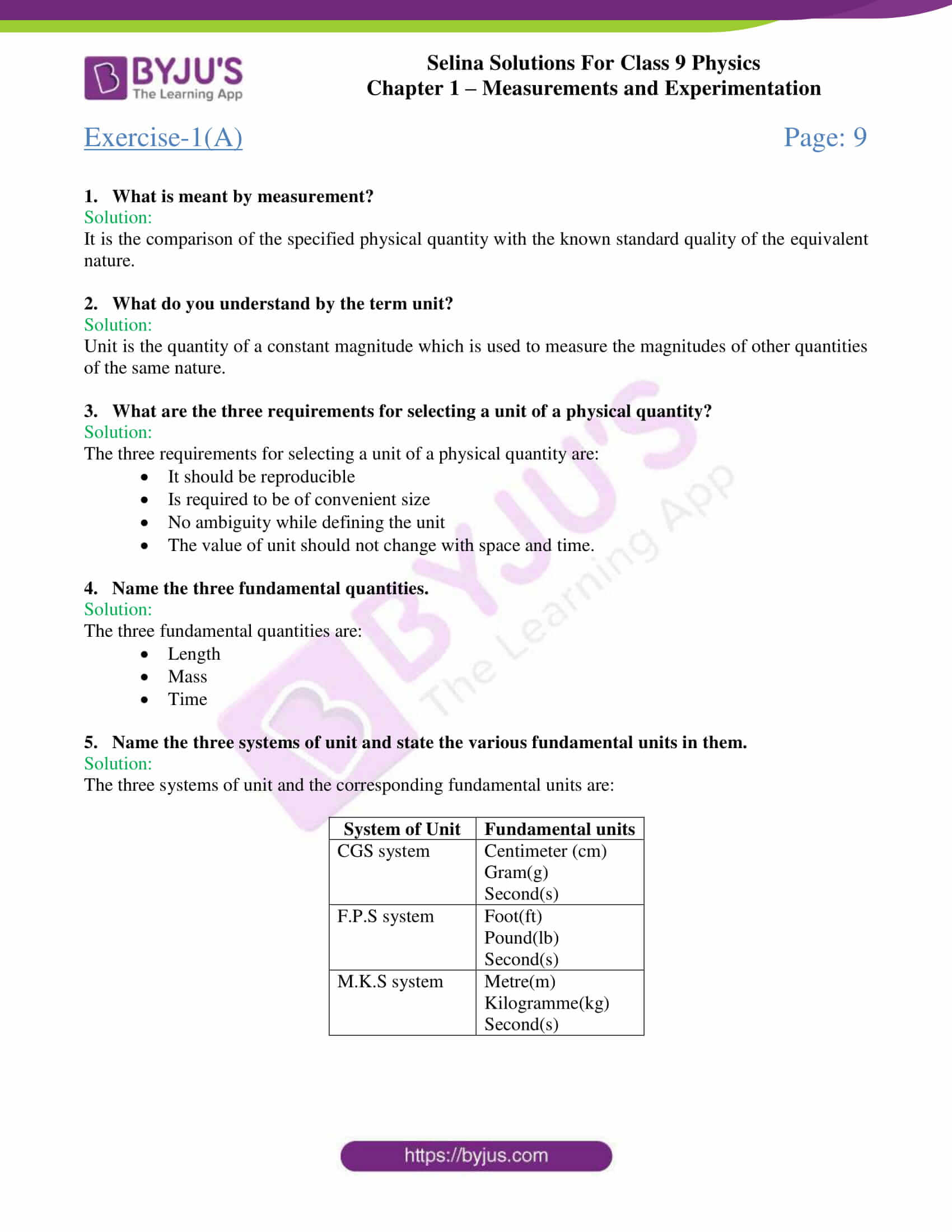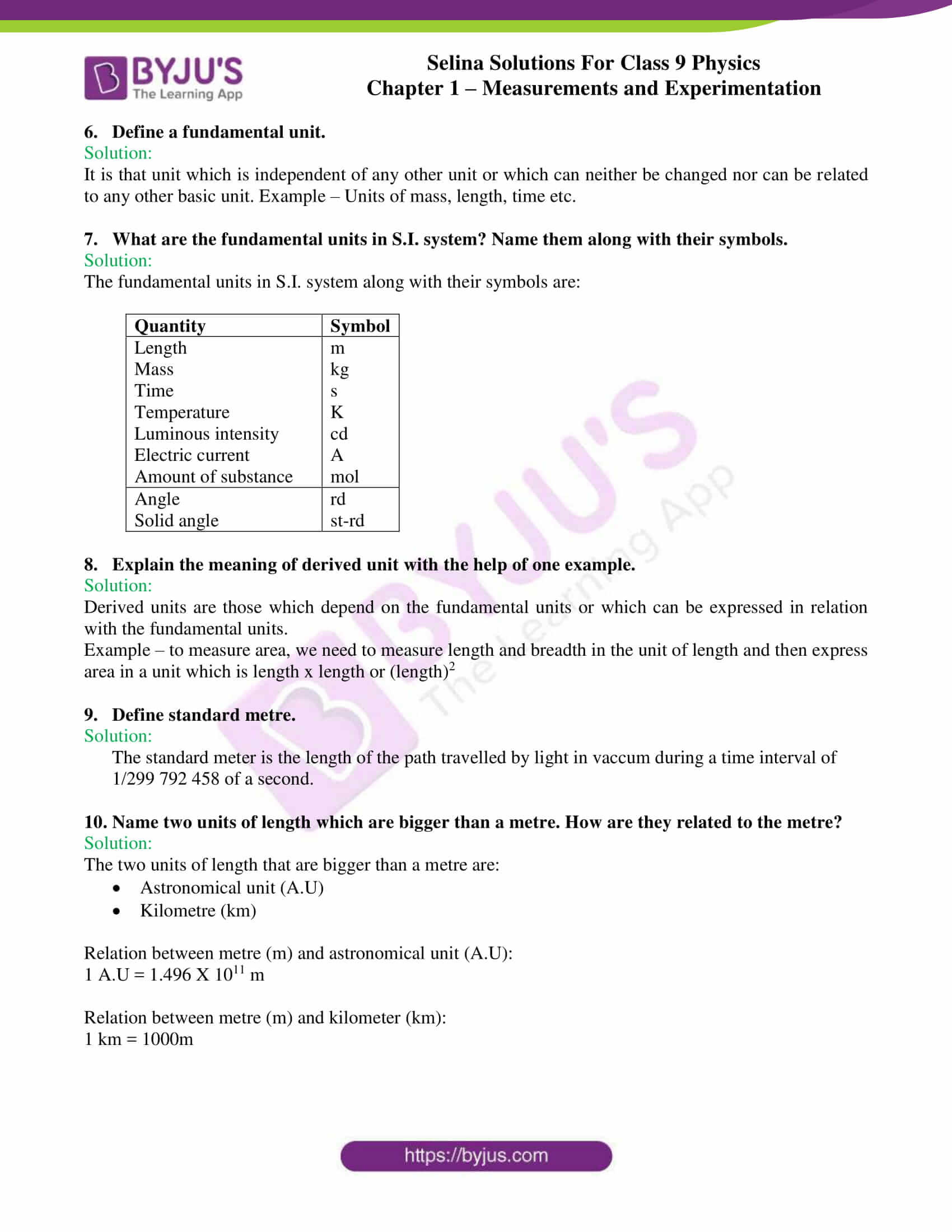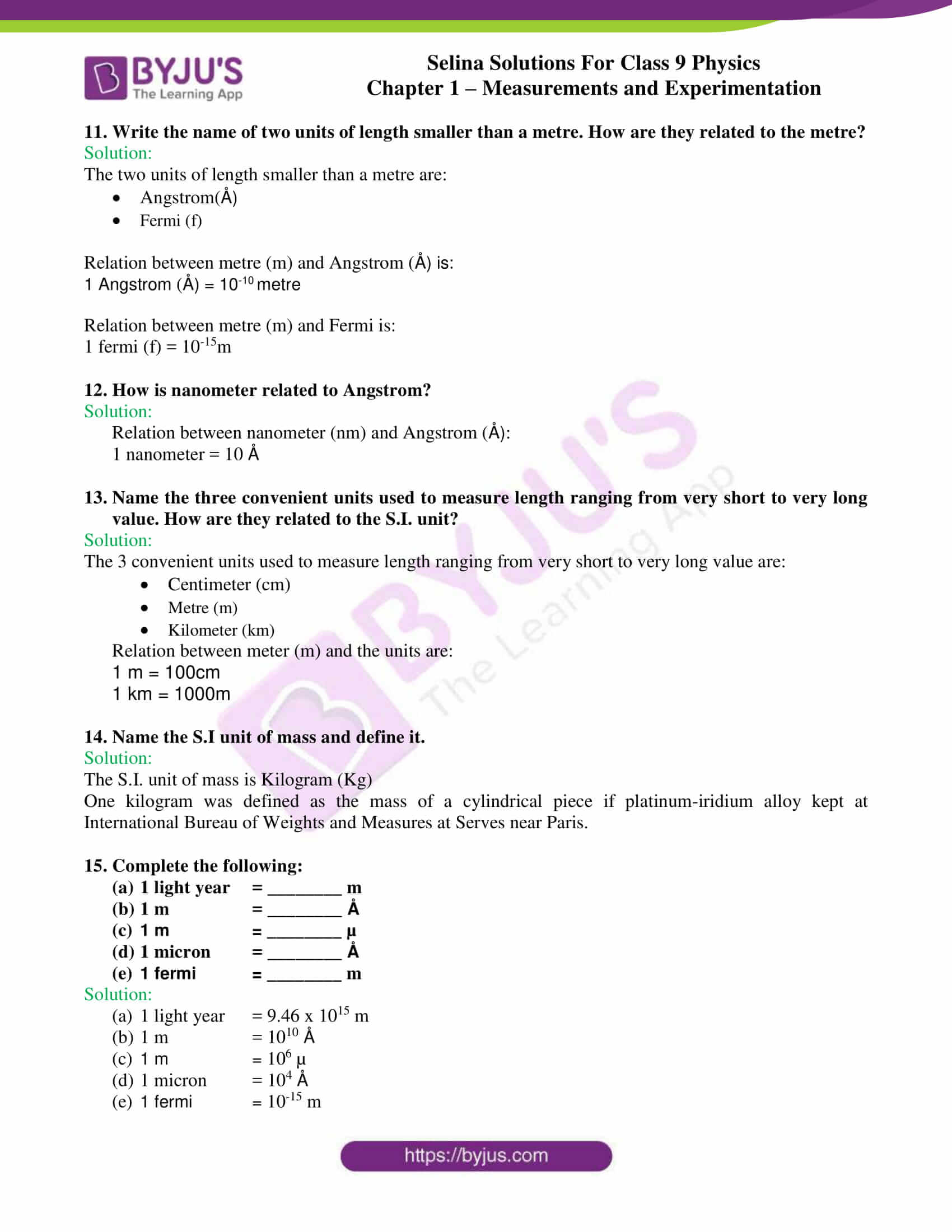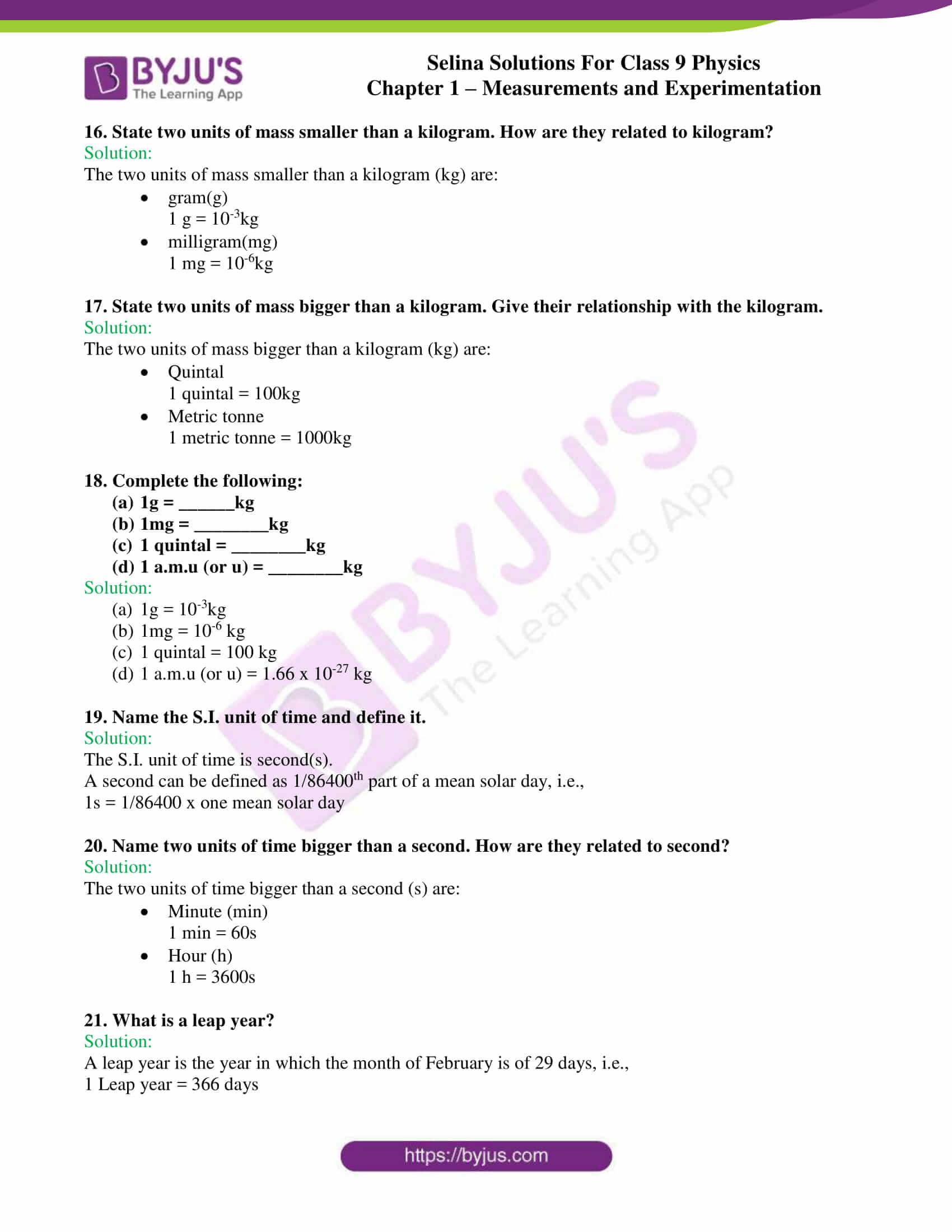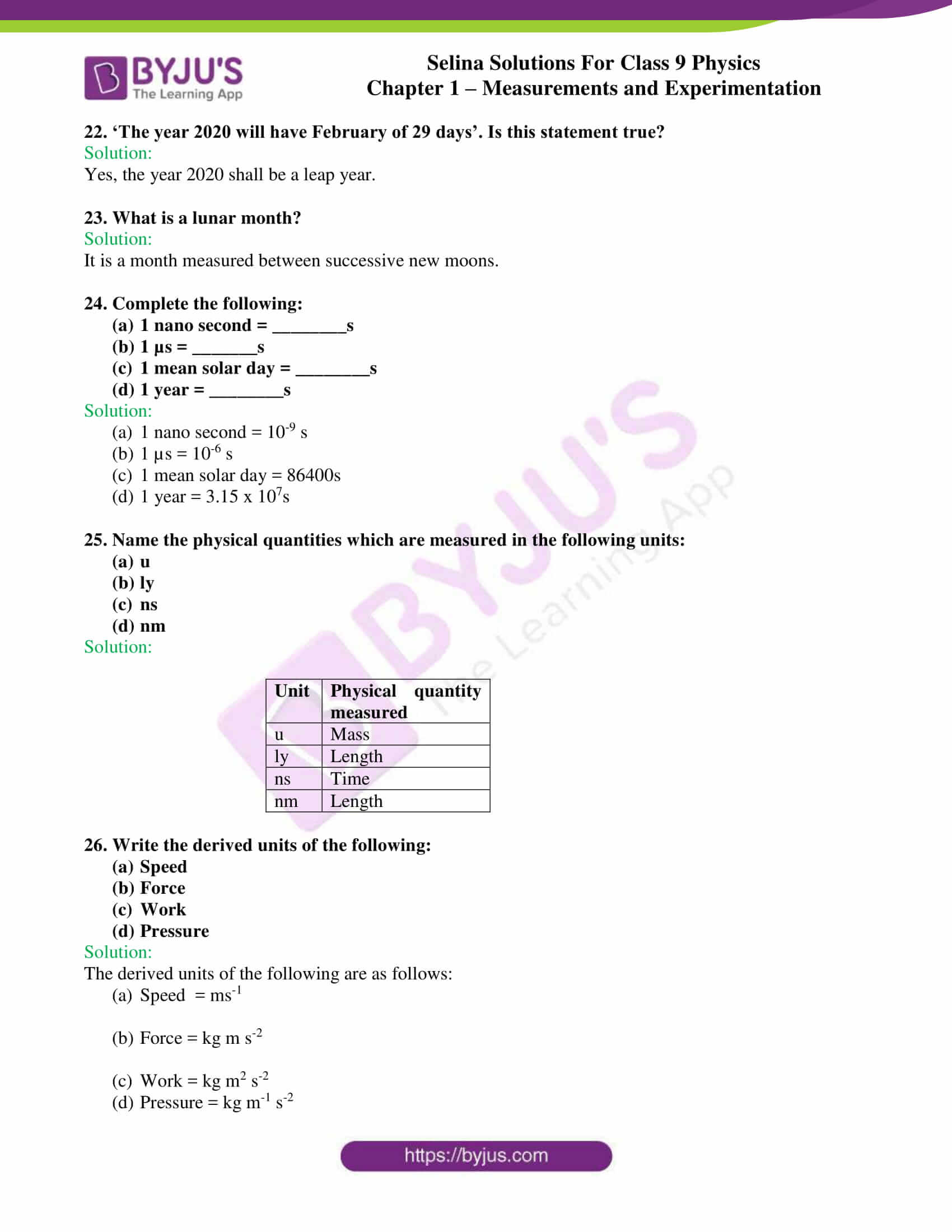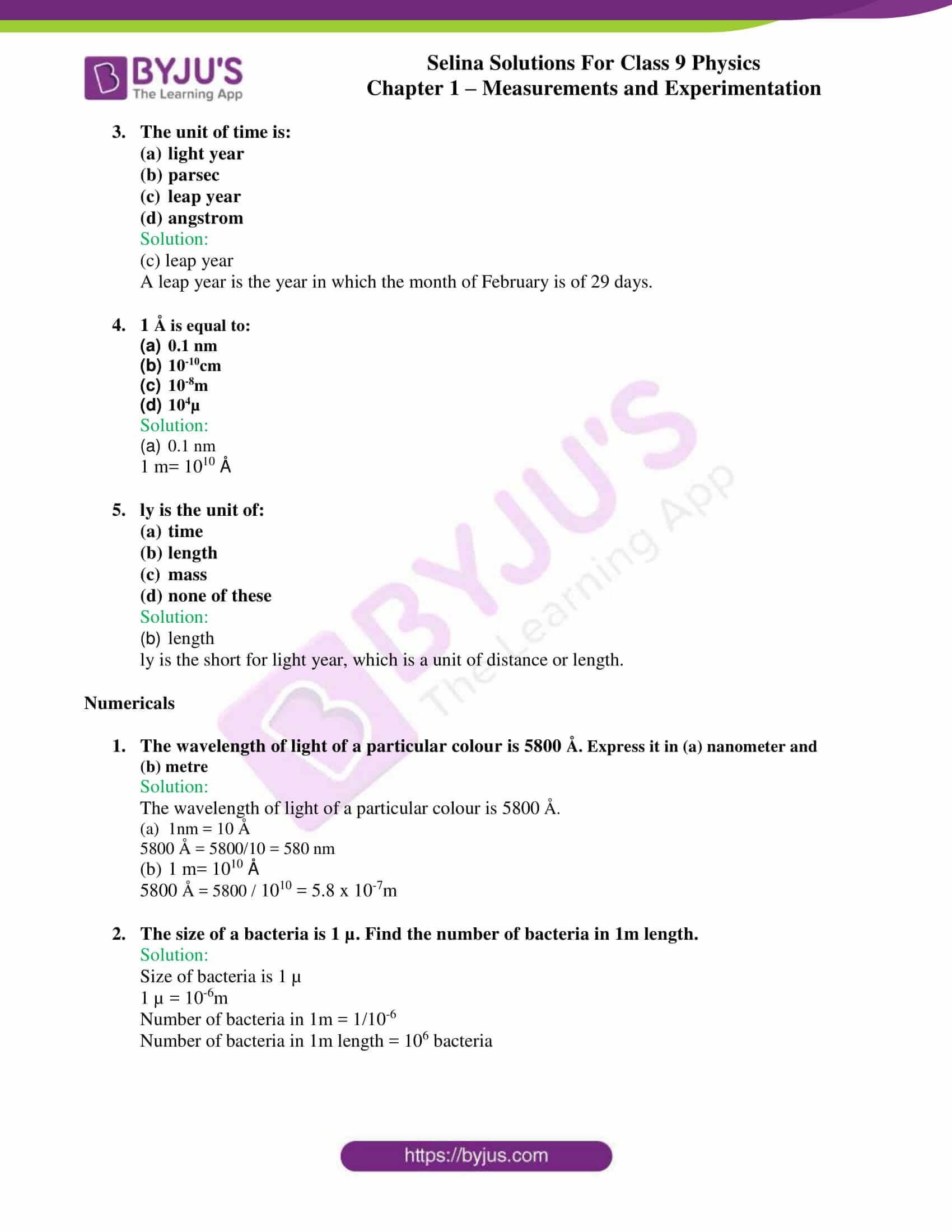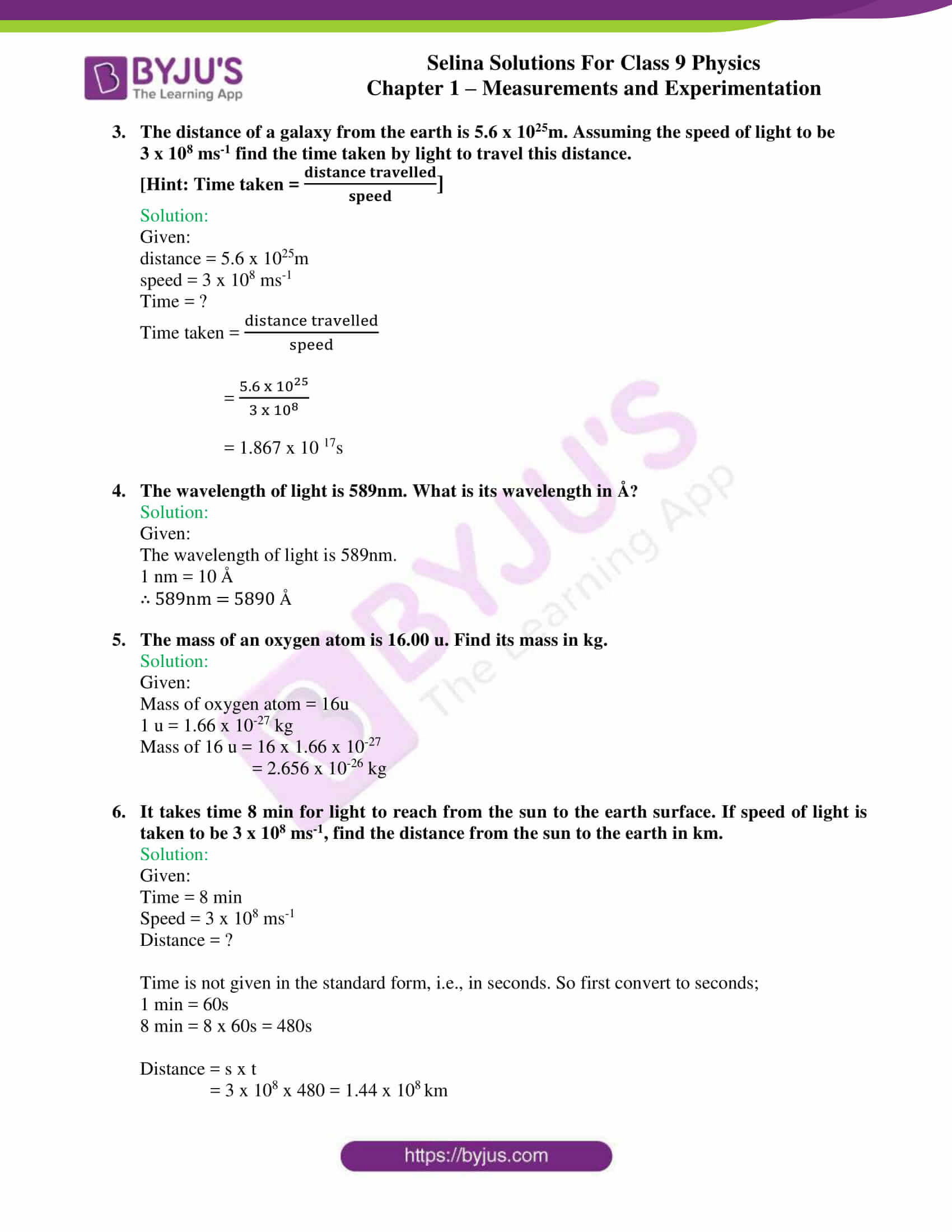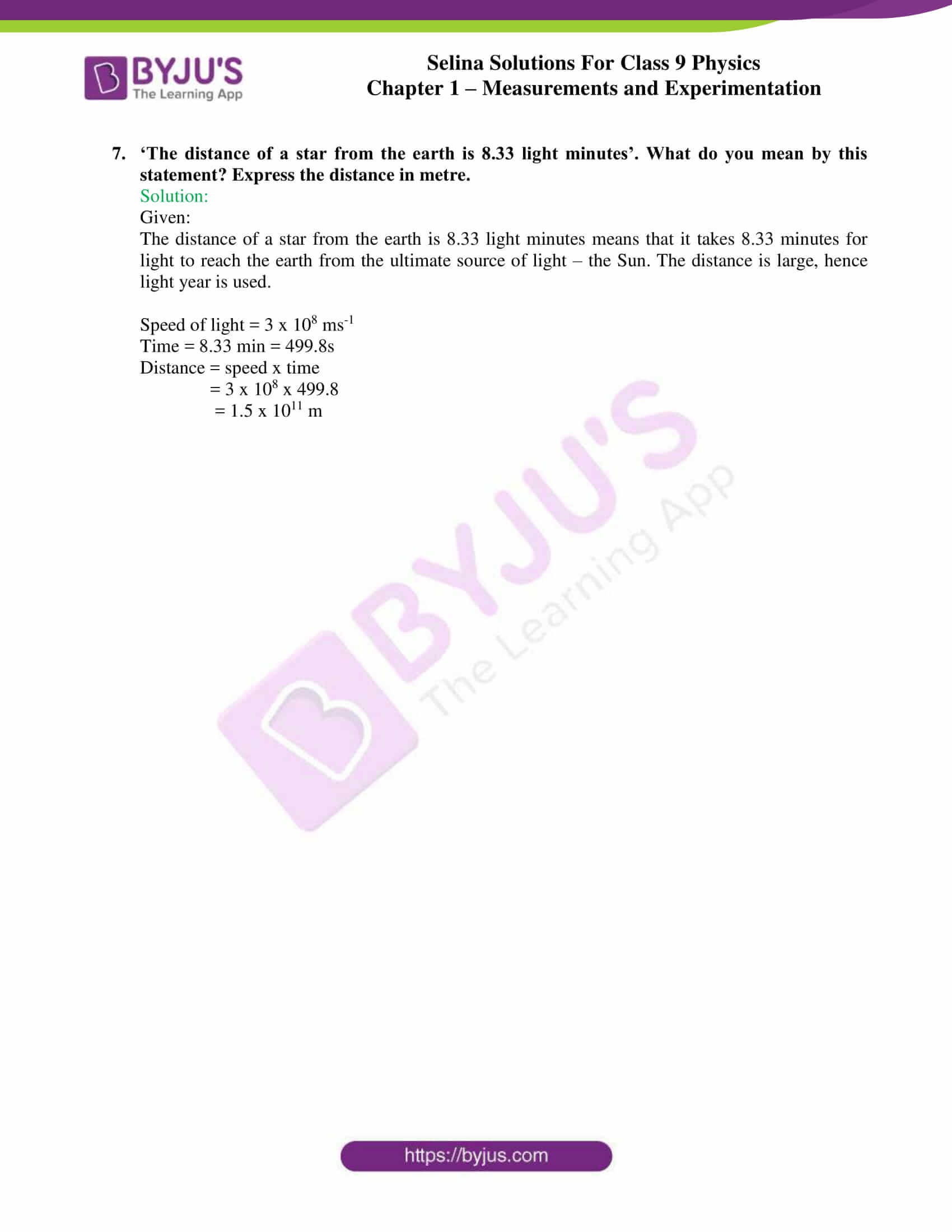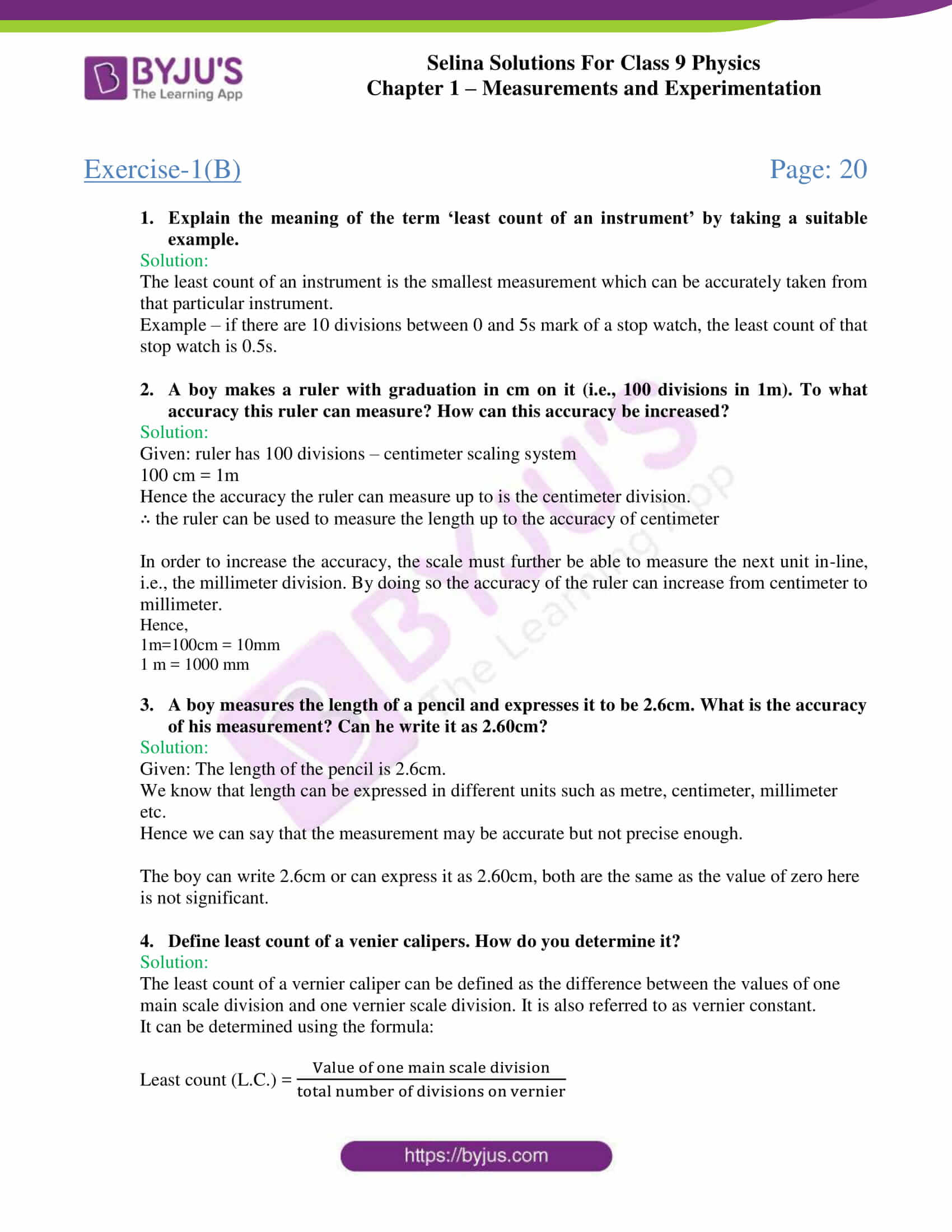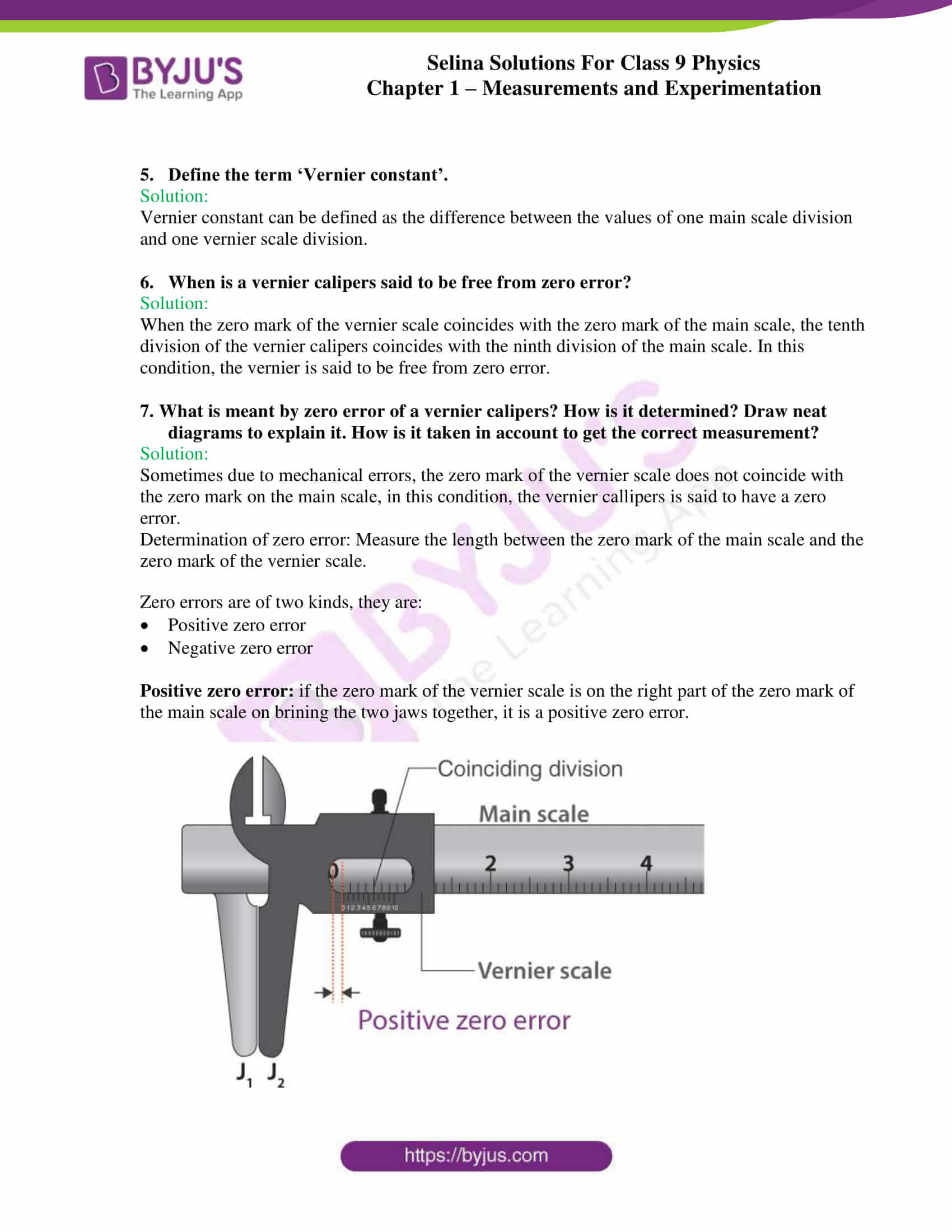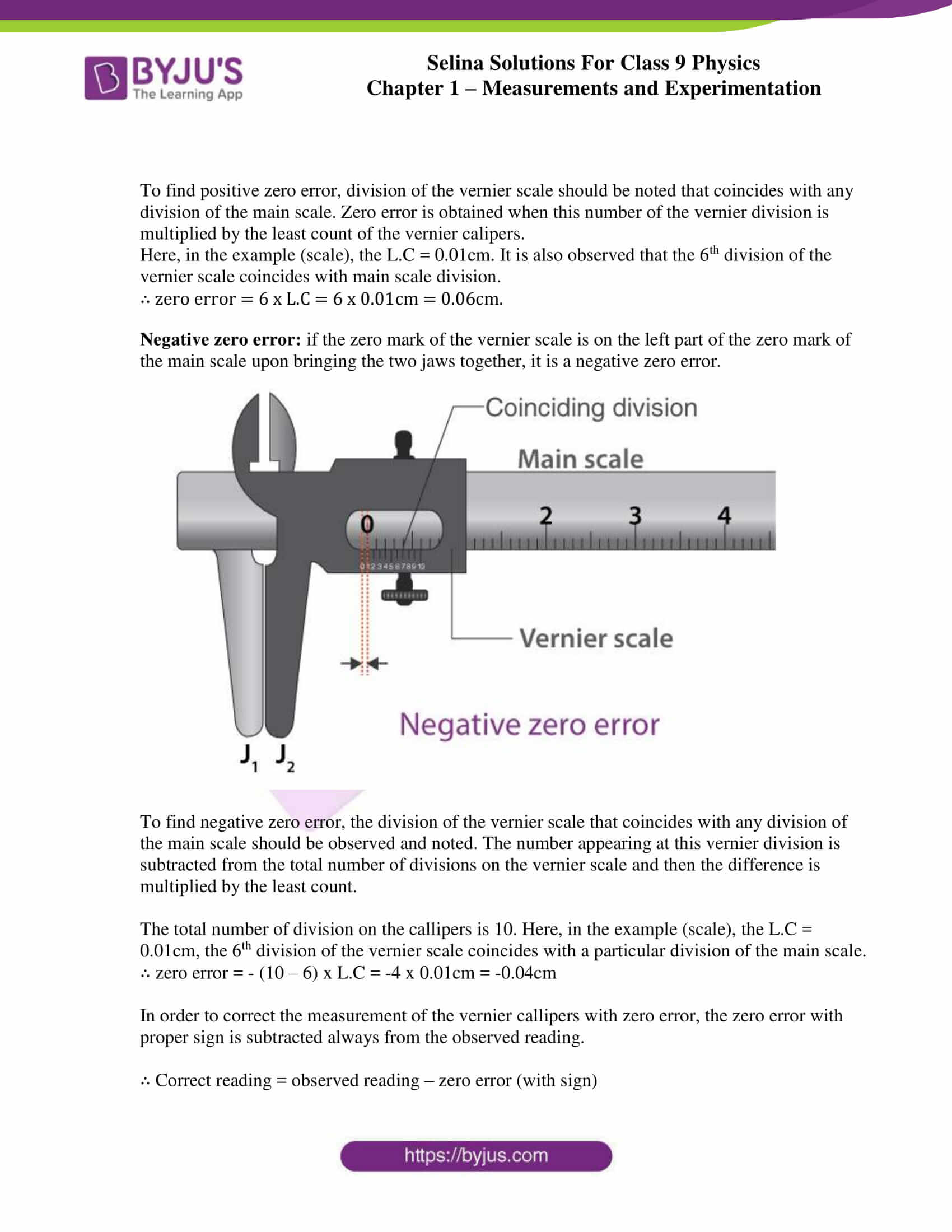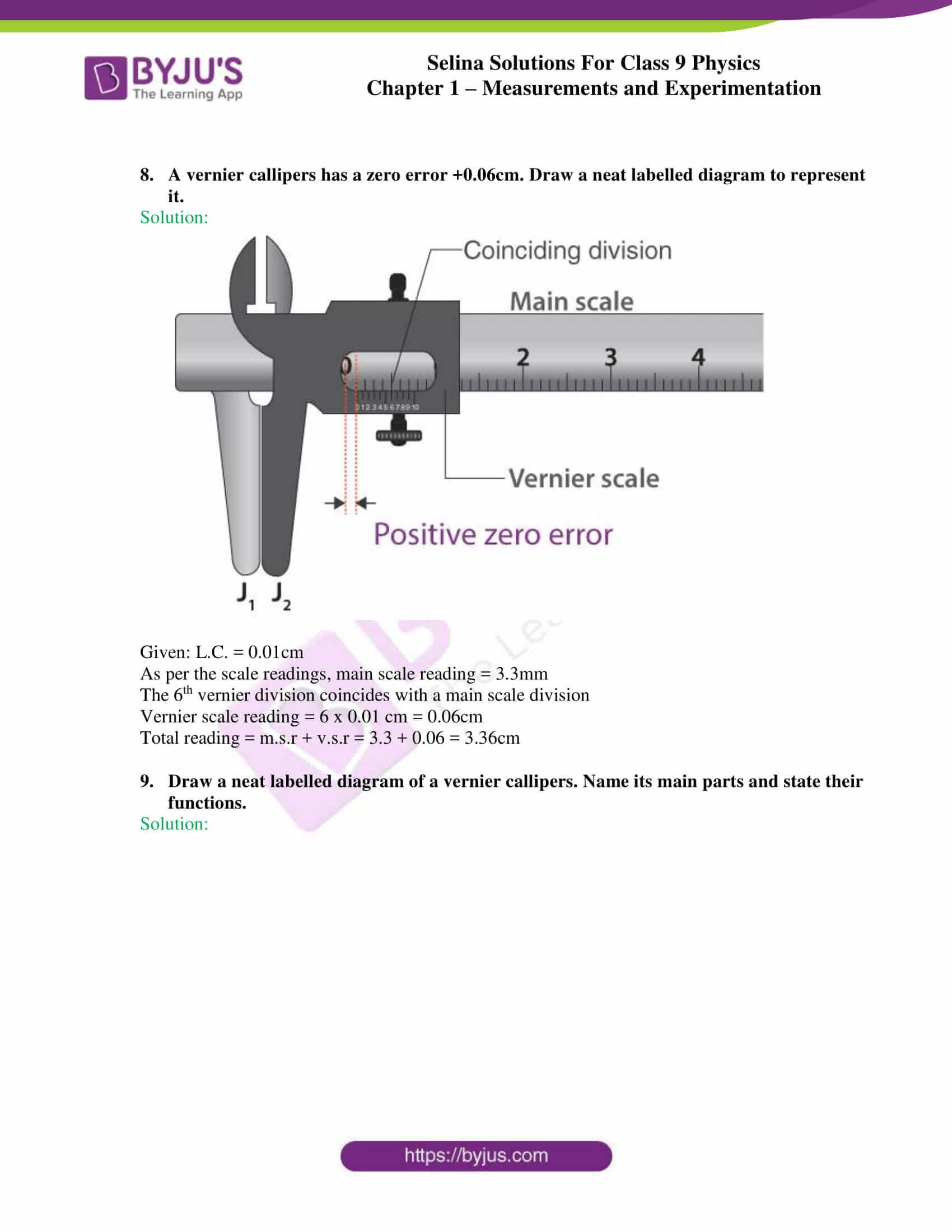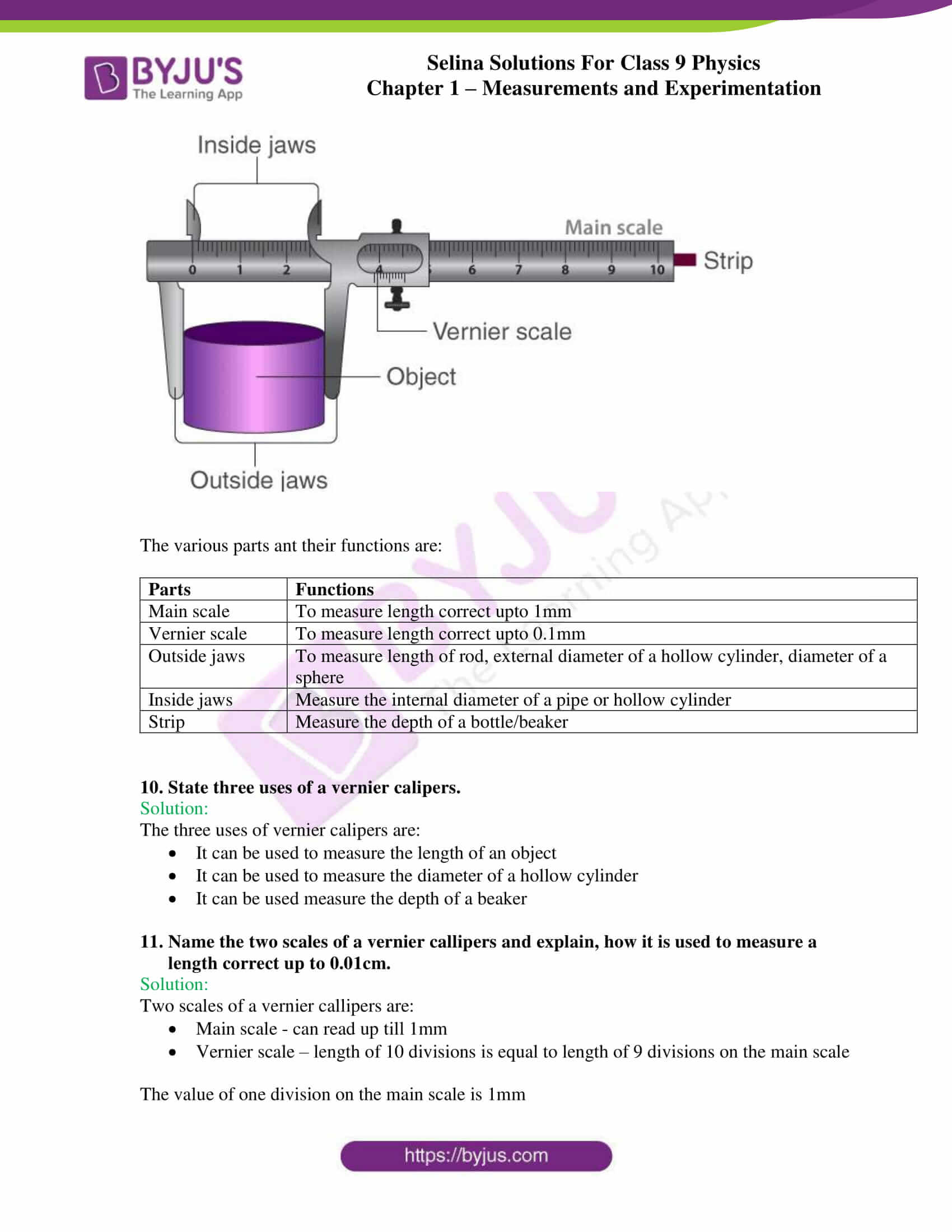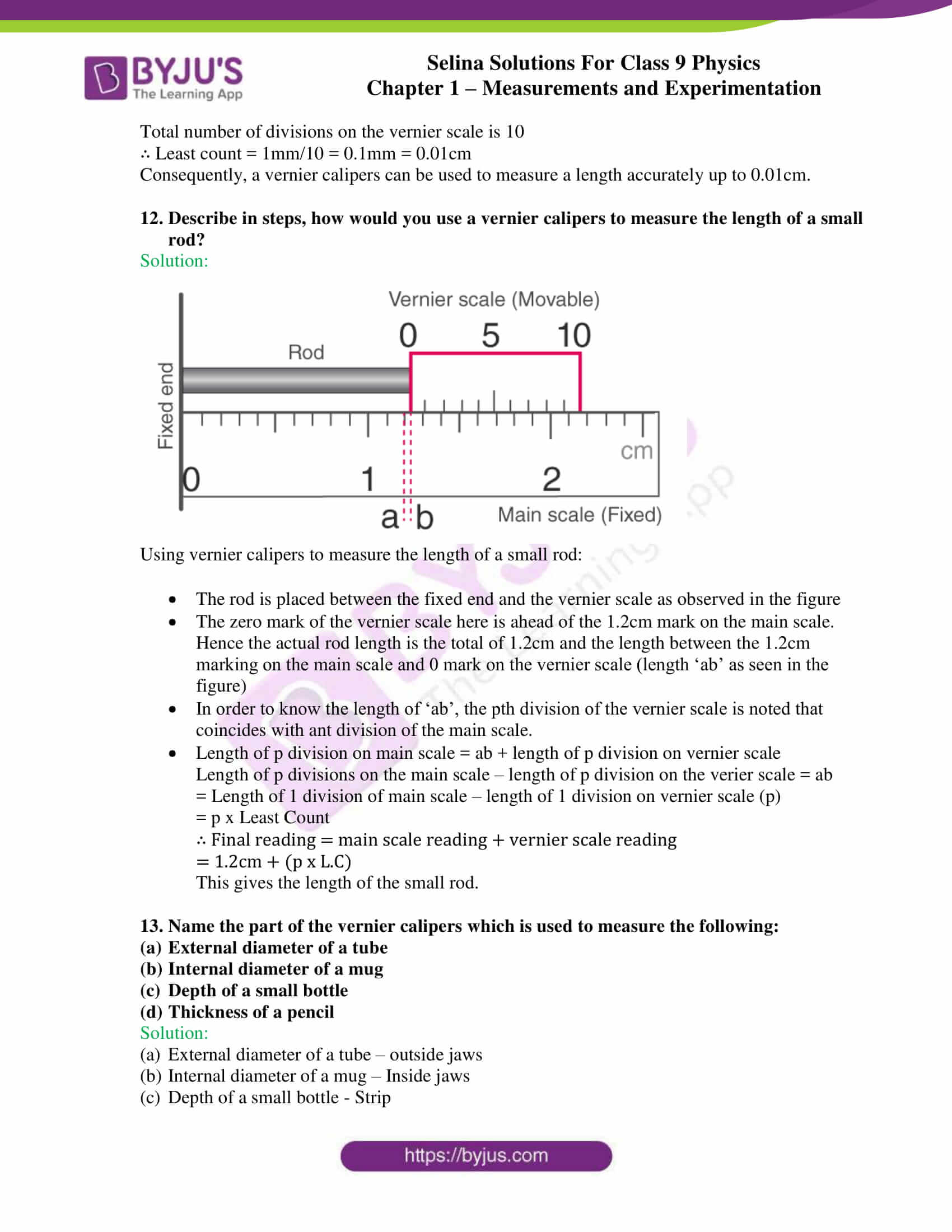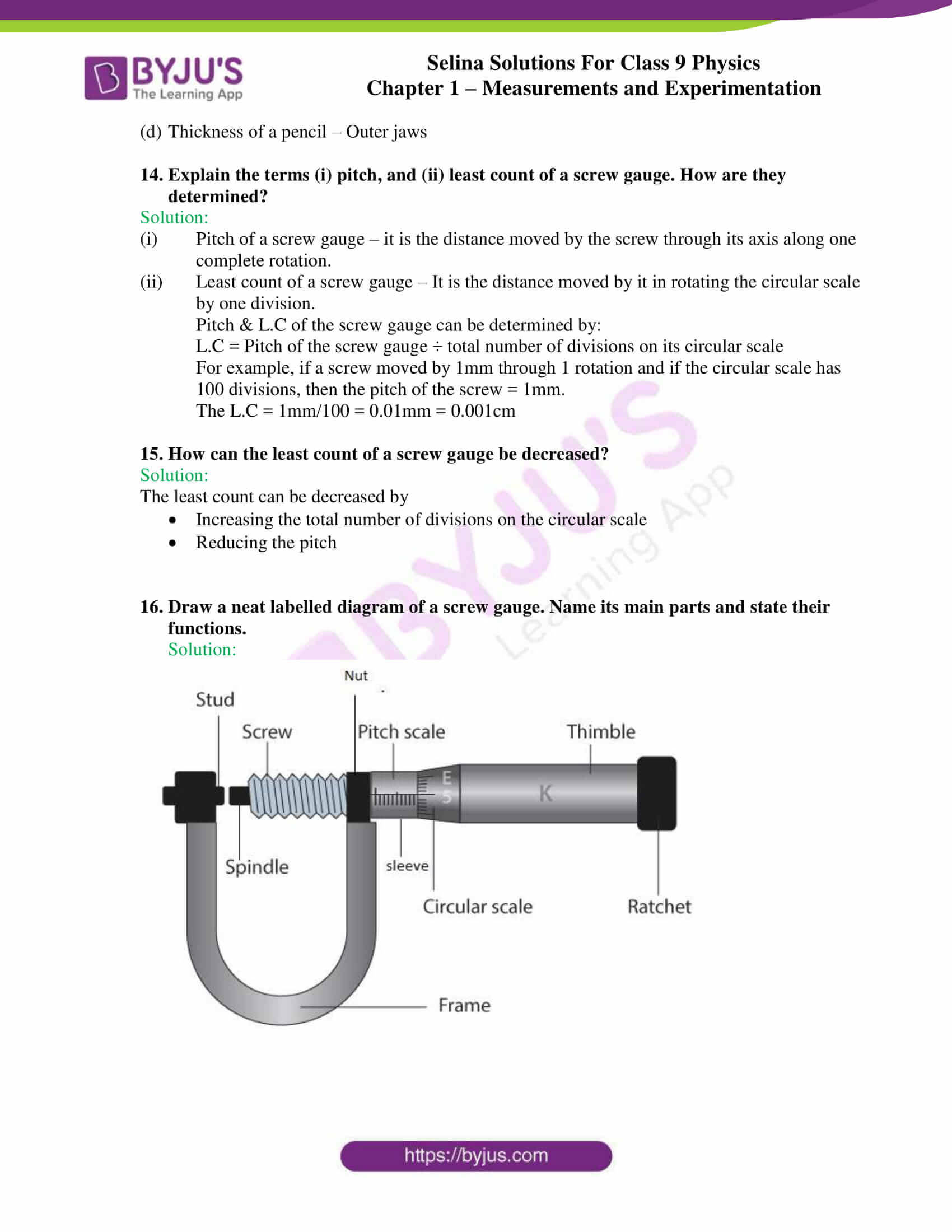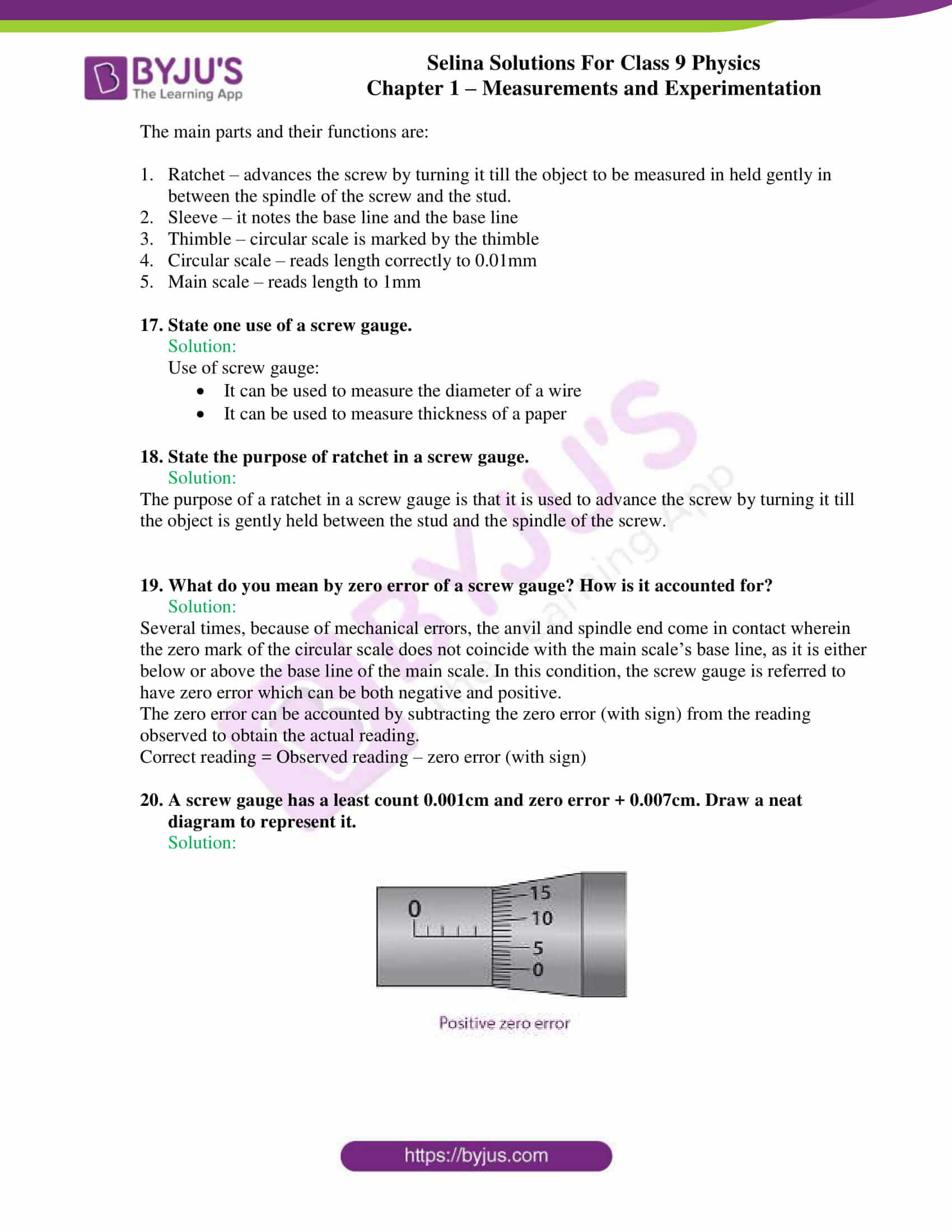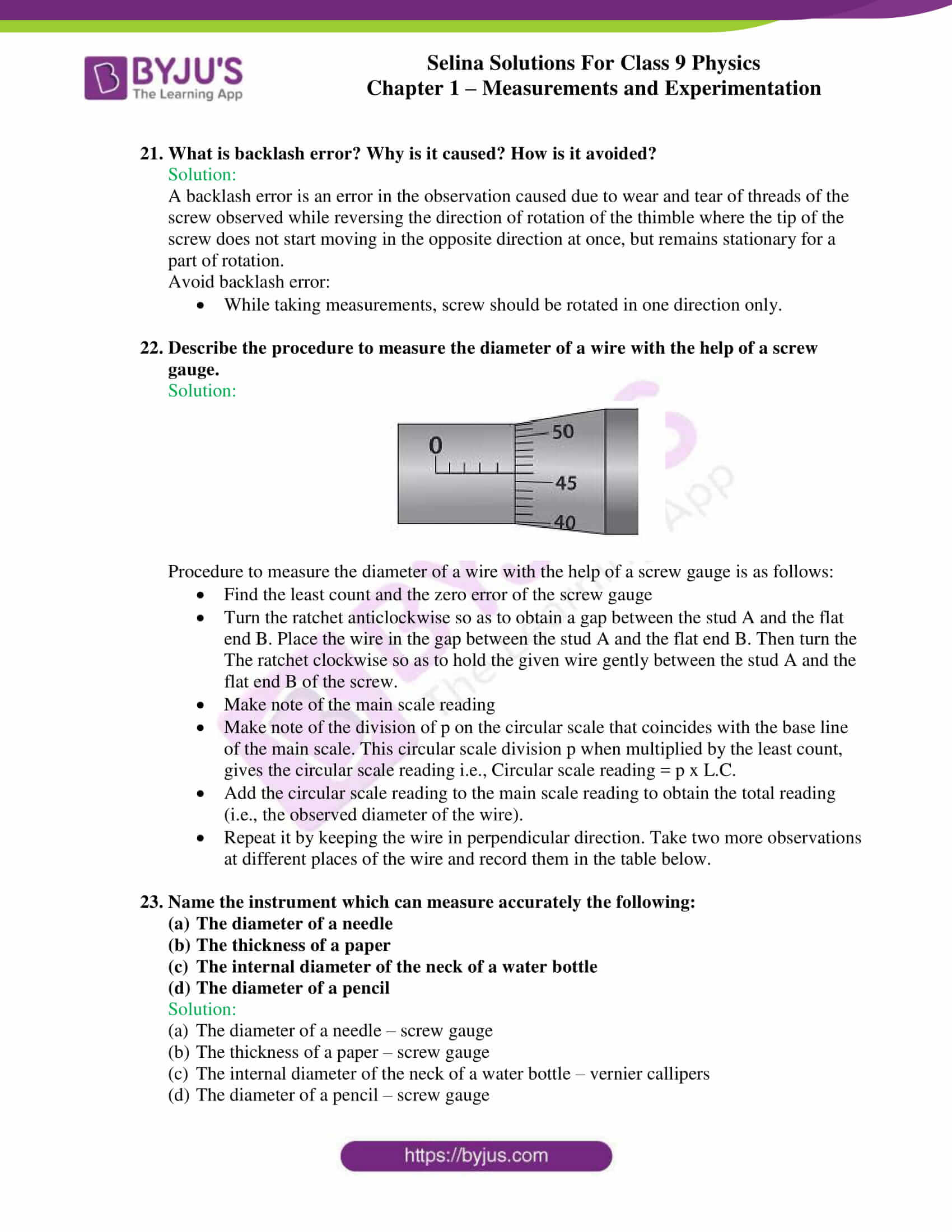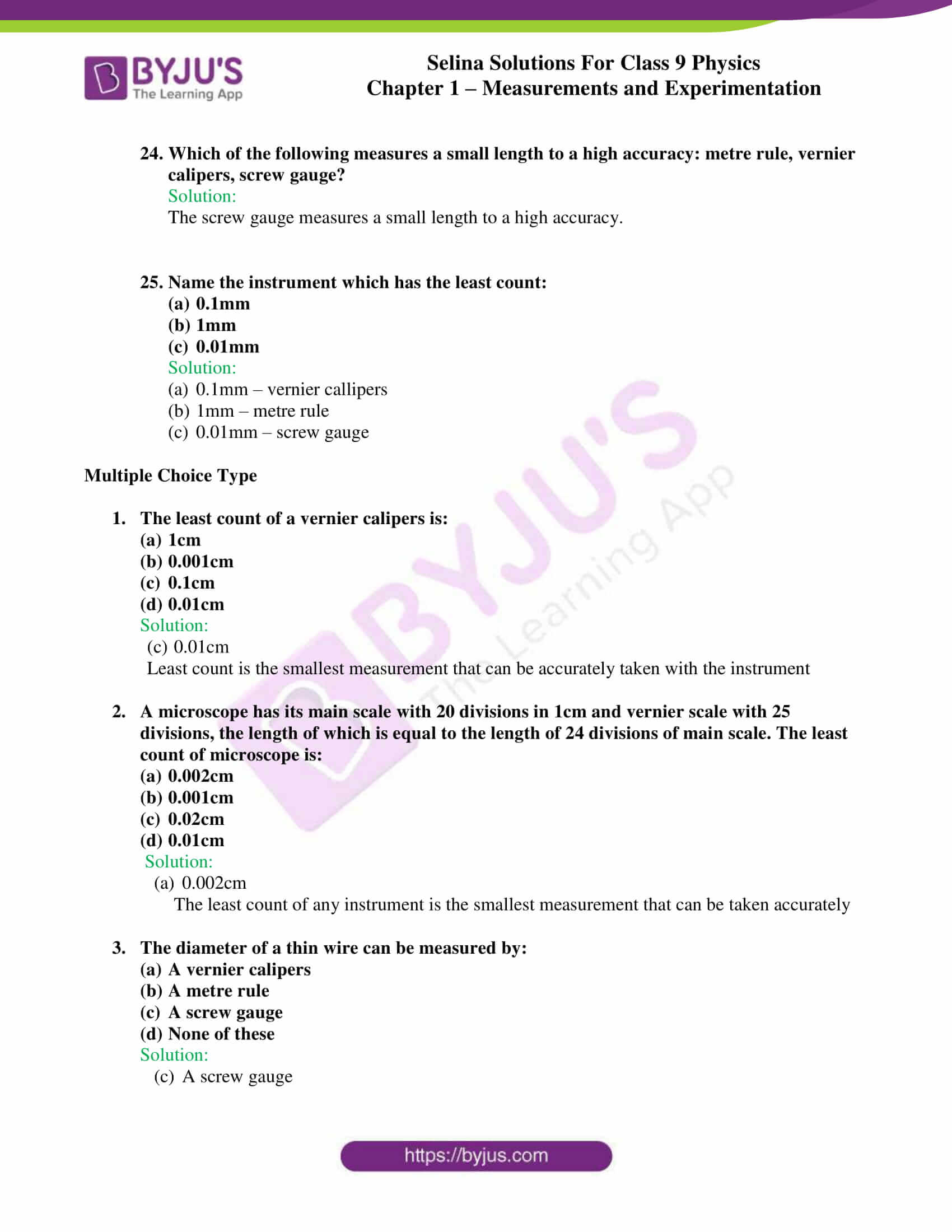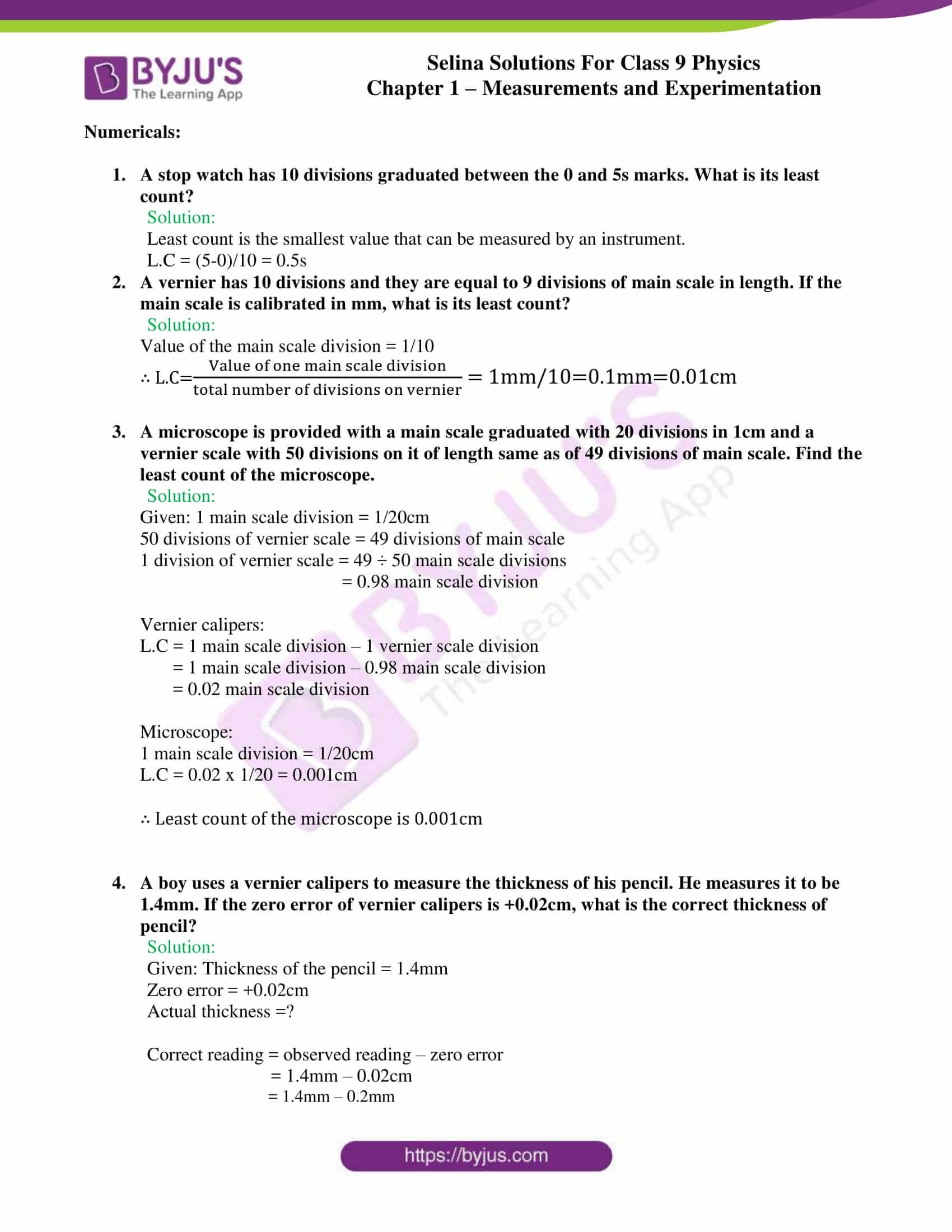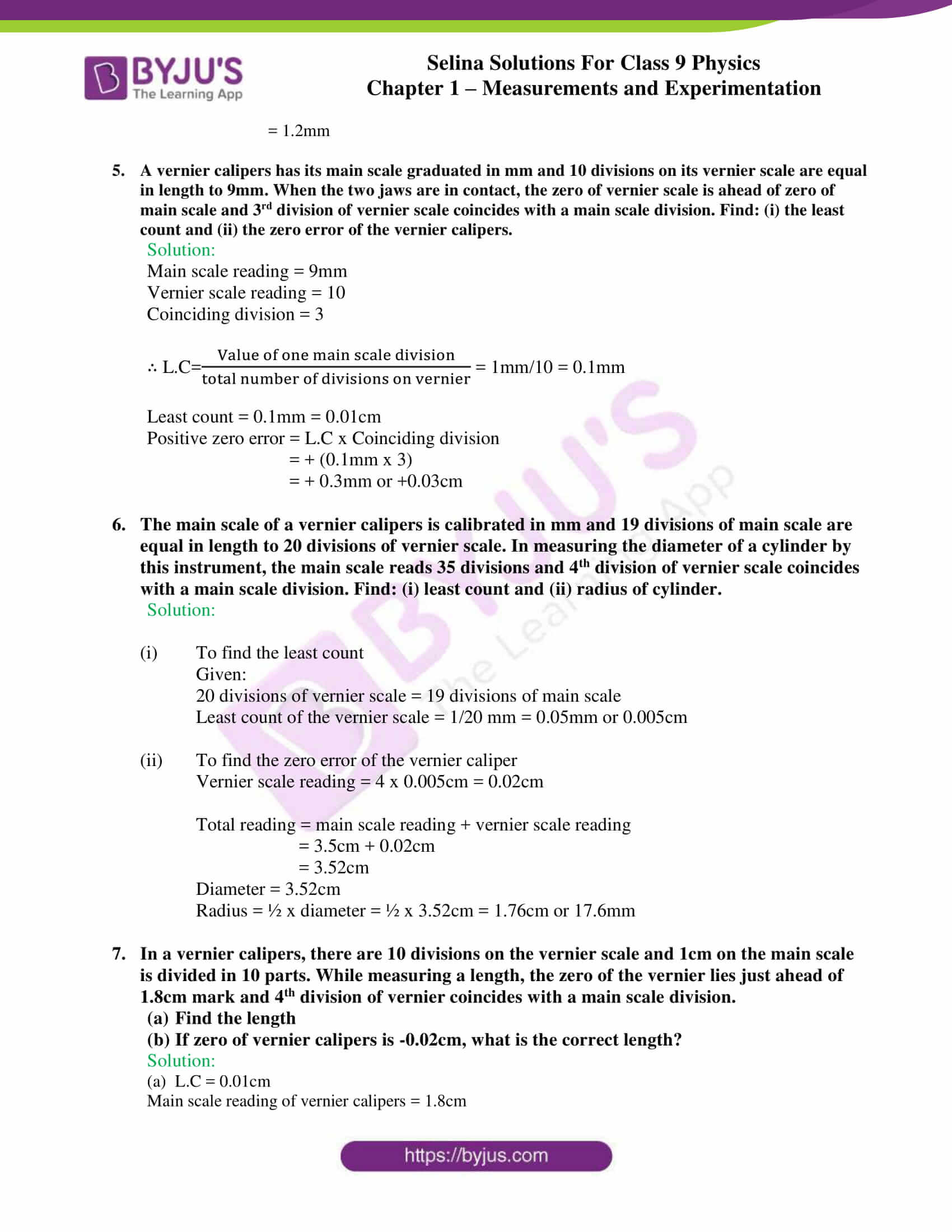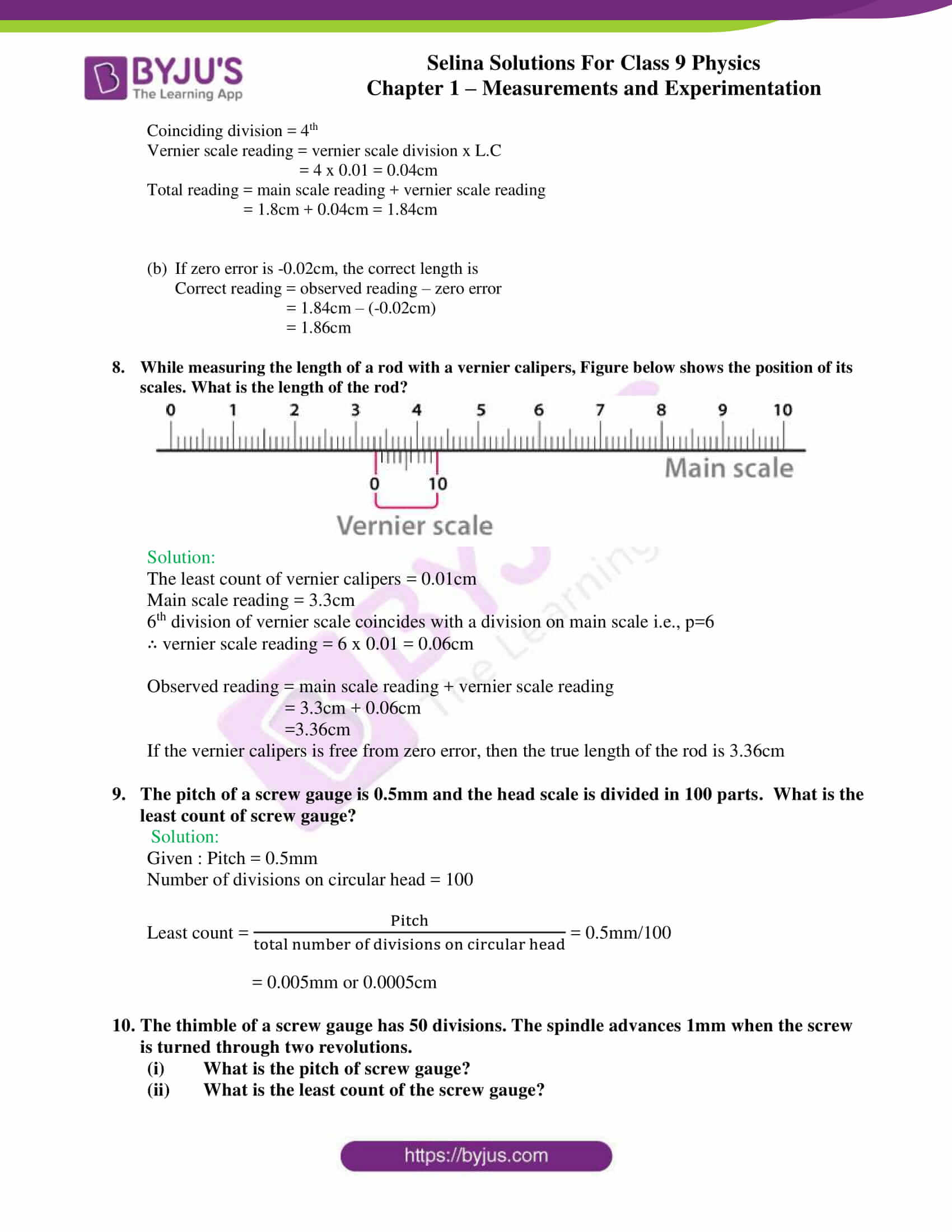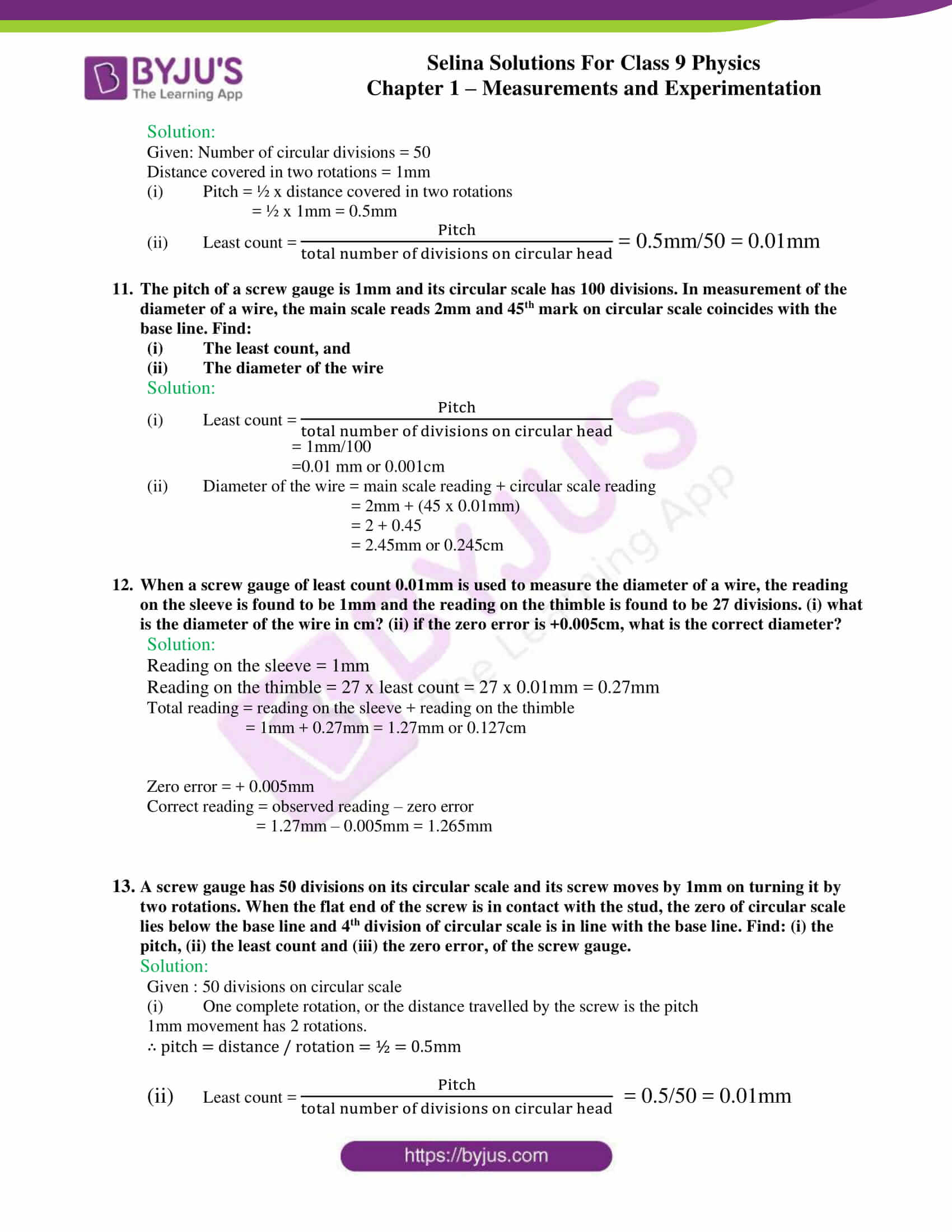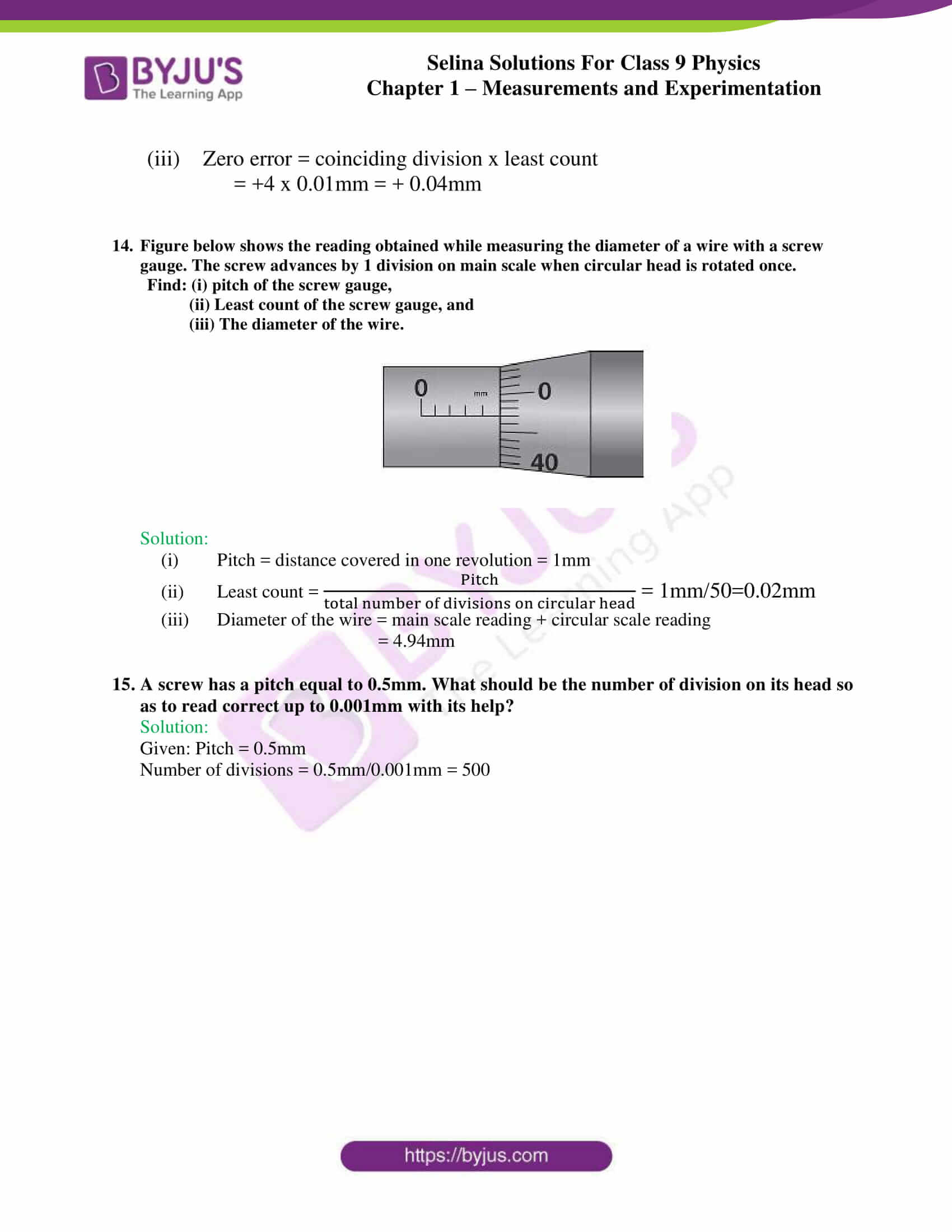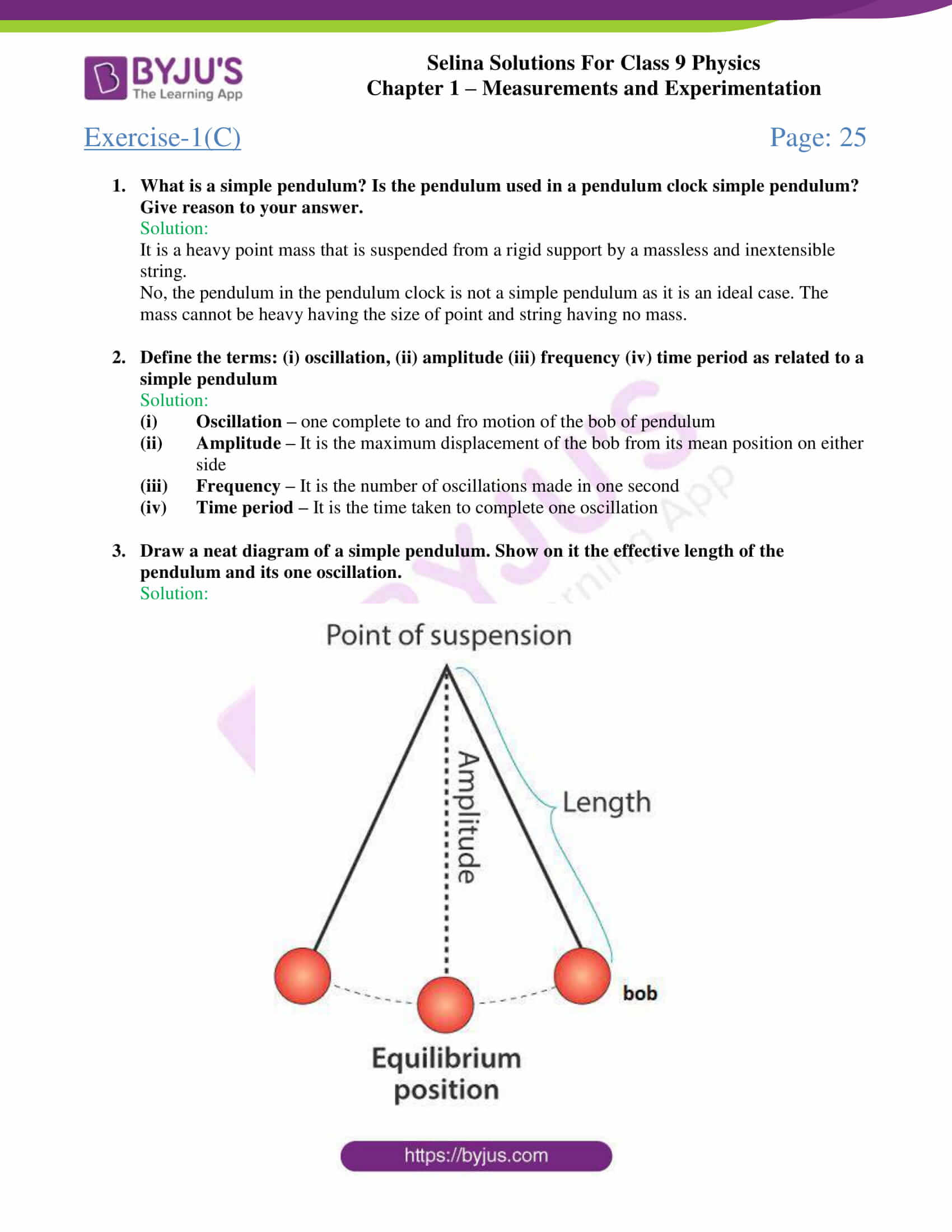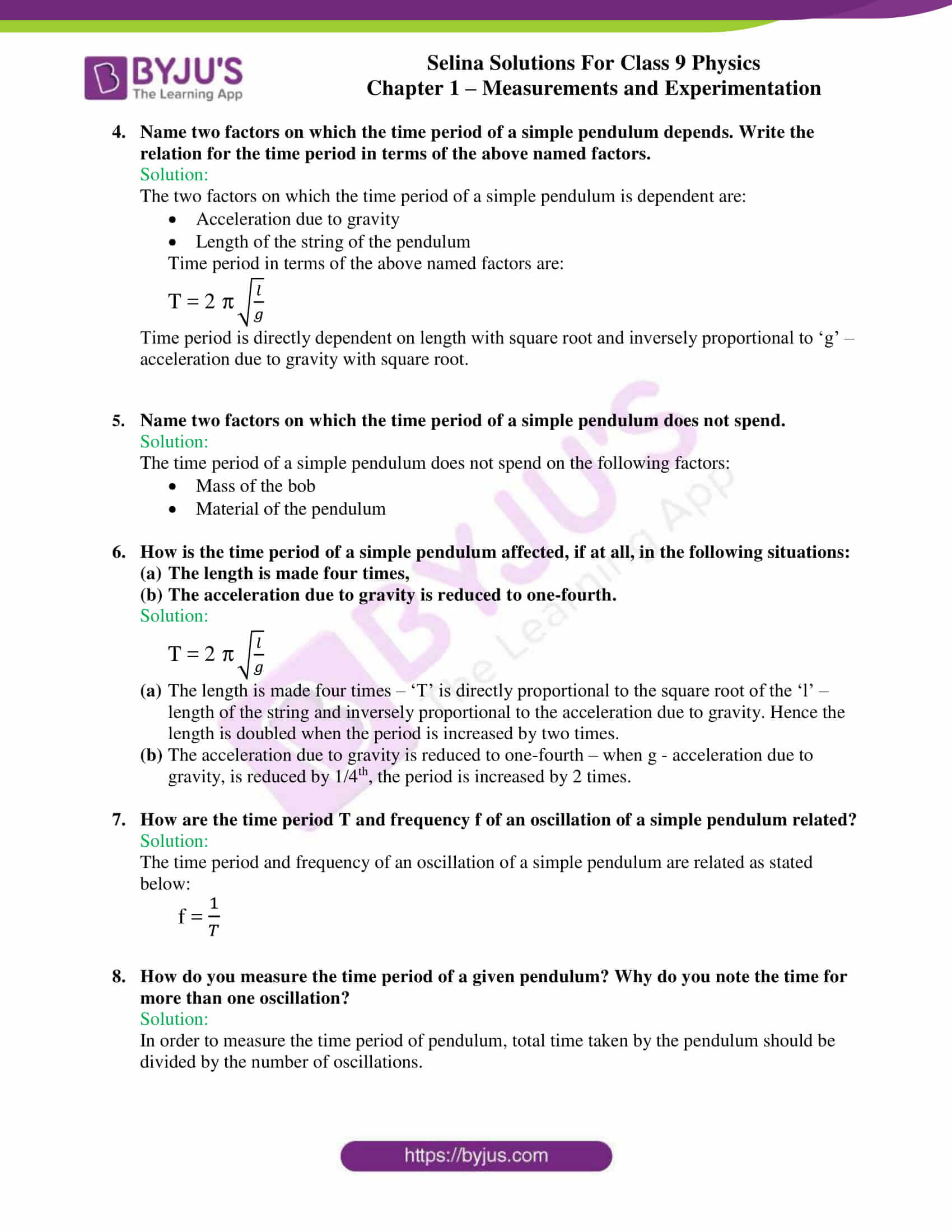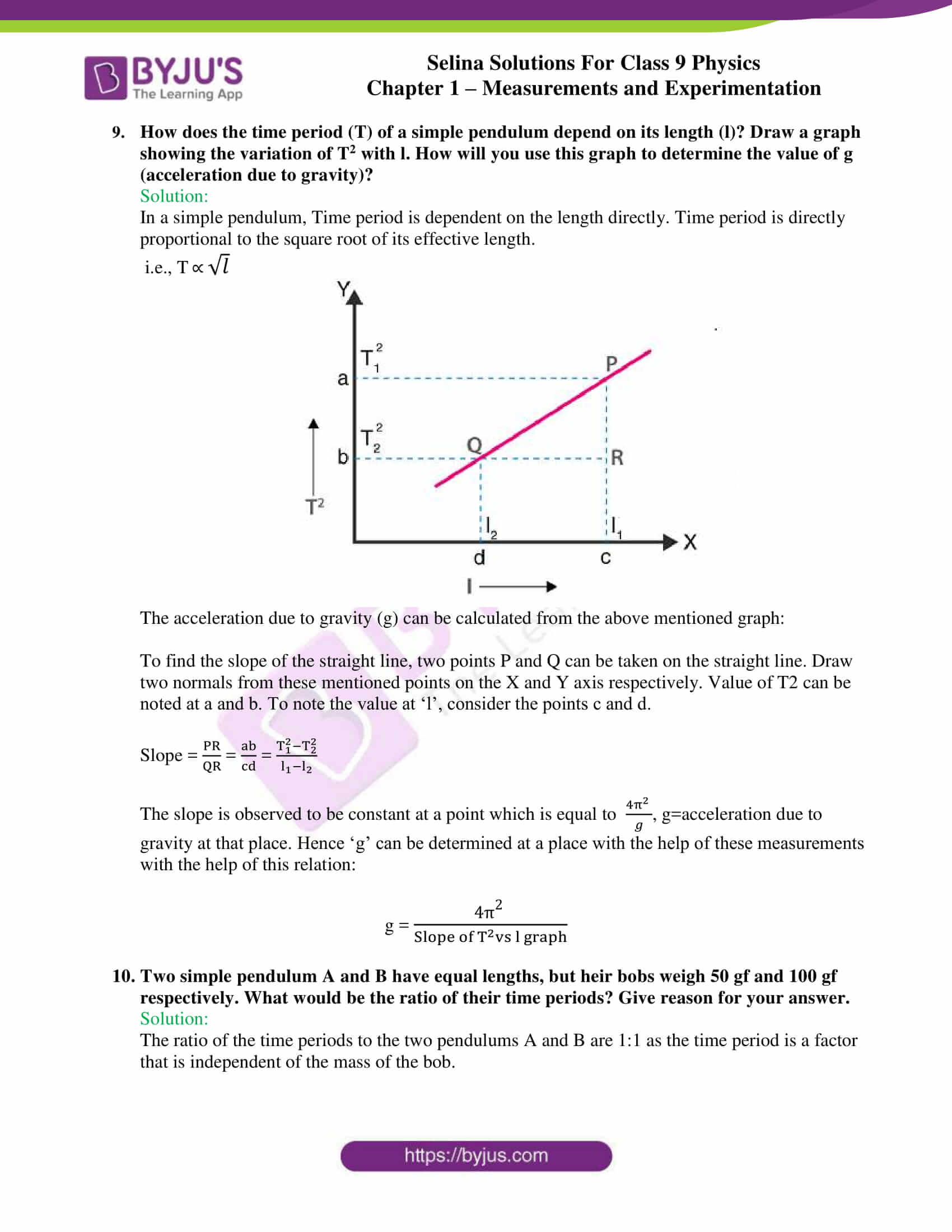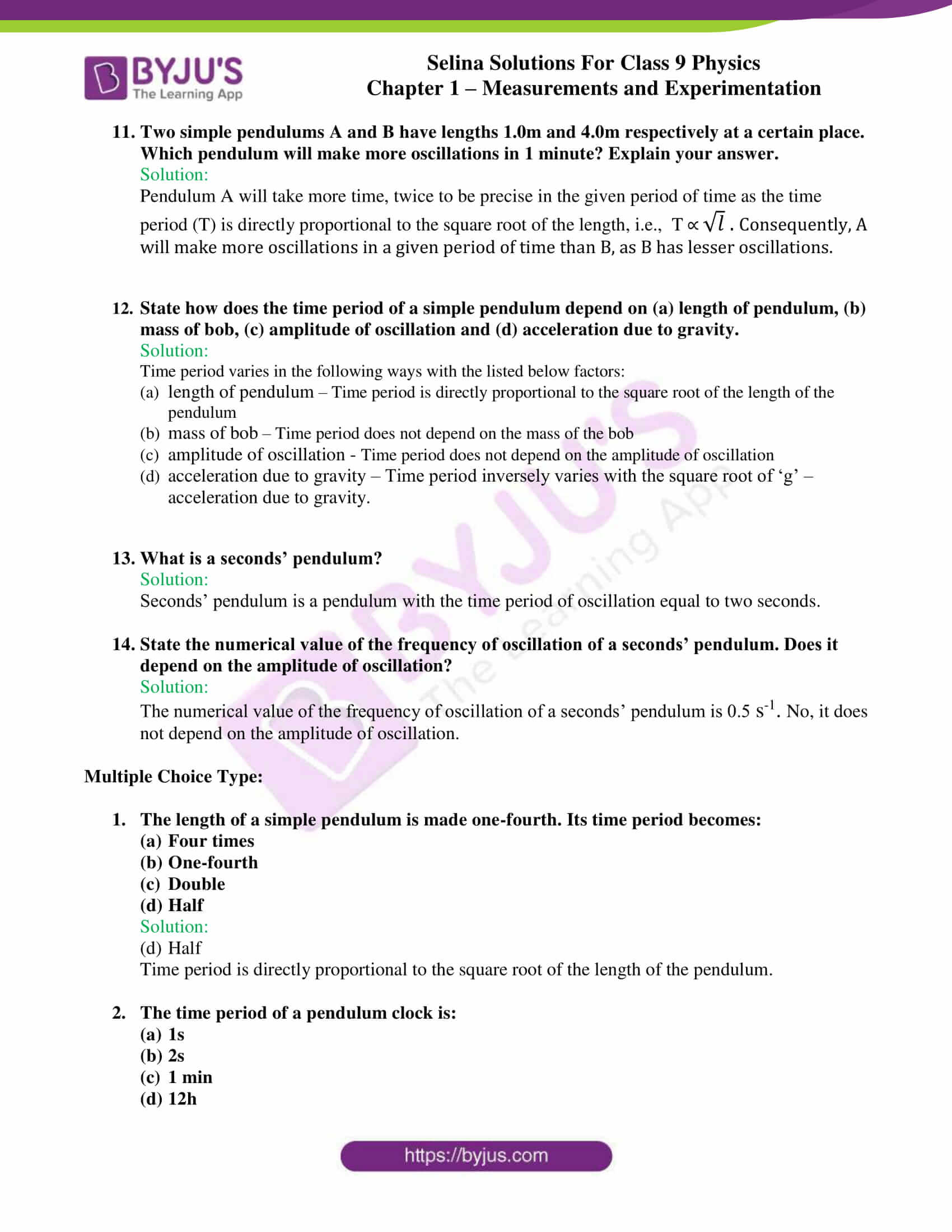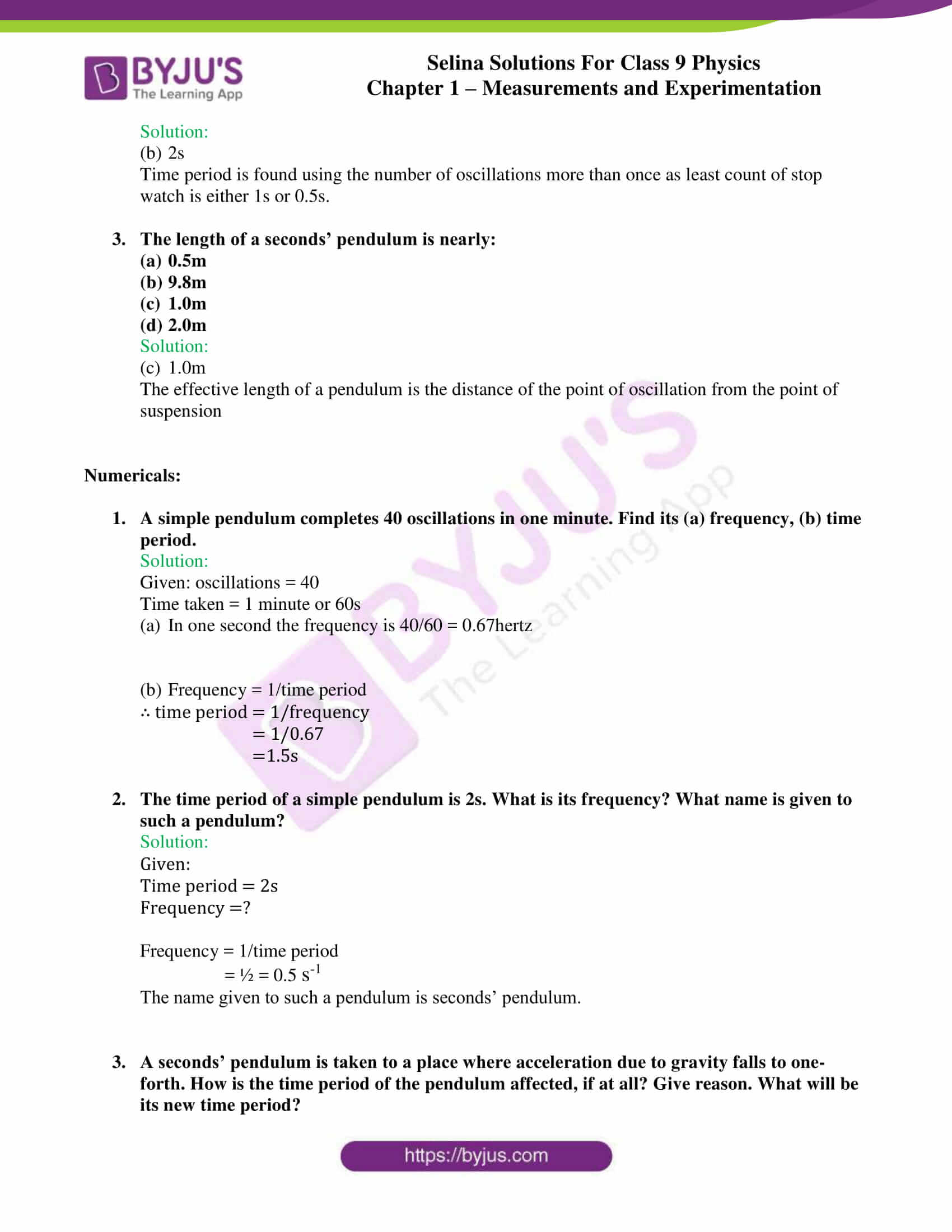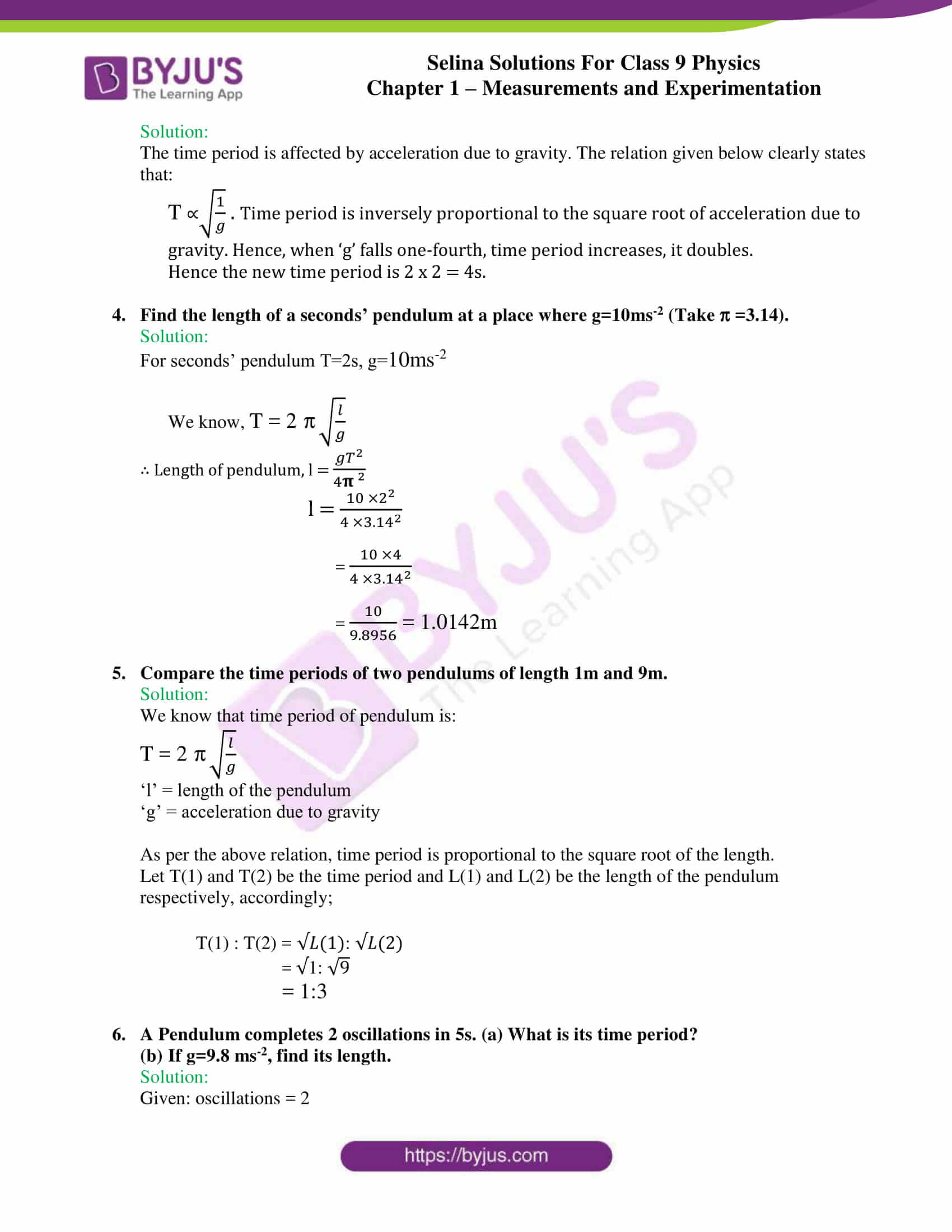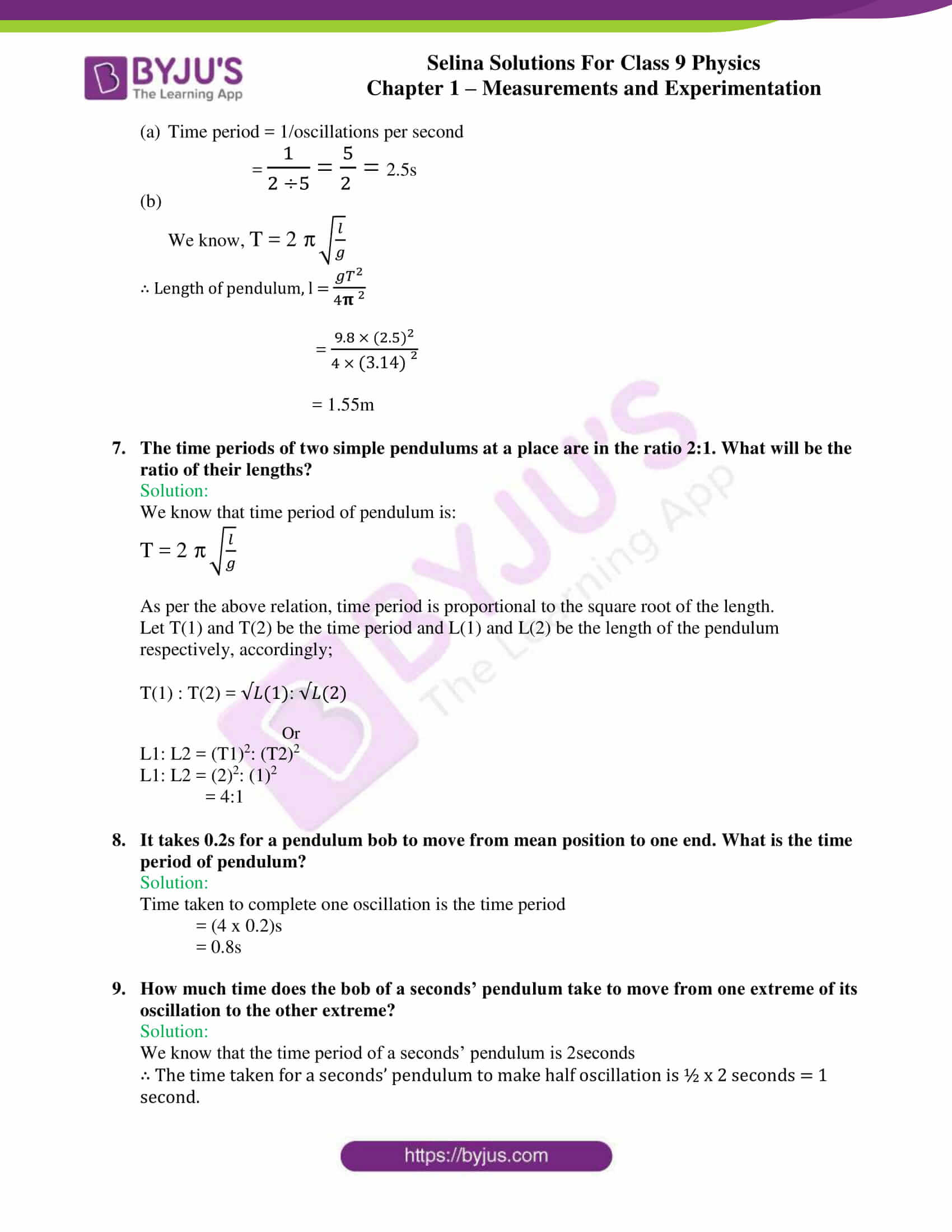## Selina Solutions for class 9 Physics Chapter 1 – Measurements and Experimentation

ICSE Class 9 Physics Chapter 1 – Measurements and experimentation are included in the syllabus for class 9 students to understand the significance of units and their association with different physical quantities.

The chapter also takes students through different instruments that can be used to measure different dimensions and the procedure of using them along with the applications of these instruments.

Measurement is the comparison of given physical quantity with the unknown standard quantity of the same nature. By the end of this chapter, students can judge different physical quantities, the suitable units etc which will be helpful for students not only from the examination point of view but also in daily life.

List of subtopics covered in Chapter 1 – Measurements and experimentation:

 Number Subtopic 1.1 Need of unit for measurement 1.2 Systems of unit 1.3 Units of length 1.4 Units of mass 1.5 Units of time 1.6 Some examples of derived units 1.7 Guidelines for writing the units 1.8 Least count of a measuring instrument 1.9 Measurement of length 1.10 Principle of vernier 1.11 Vernier Callipers 1.12 Principle of a screw 1.13 Screw Gauge 1.14 Measurement of time

List of Exercise

 Name of the exercise Number of questions Page number Exercise-1A 40 9 Exercise-1B 43 20 Exercise-1C 26 25

## Selina Solutions for Class 9 Physics Chapter 1 – Measurements and Experimentation

In this chapter, we get enlightened about the most fundamental topic in physics, measurements and experimentation. Physics is a natural science that deals with the nature, behaviour and properties of energy and matter. It requires experiential learning that involve experimentation. To gauge different physical quantities that we study in physics, a complete understanding of different units is essential. Unit is used to measure the magnitudes of different quantities of the same nature.

The chapter discusses the international system of units – the symbols and expression of these units for different physical quantities, and other systems of measurements such as C.G.S and F.P.S are also discussed.

Students are also enlightened with practical aspects of this unit such as the use of screw gauge and vernier callipers for the measurement of various physical quantities such as the thickness of a wire, the radius of cylinders etc. The chapter also discusses time and simple pendulum and various numerical are also solved on the same topic. Towards the end of the explanation, numerical are solved, along with examples so that students understand the calculation and expression.

### Key Features of Selina Solutions for class 9 Physics Chapter 1 – Measurements and experimentation

• Selina solutions enable students for the preparation of class 9 examinations stress-free
• Solutions are answered with the help of relevant analogies
• Tables are used wherever necessary to remember and learn concepts easily
• Solutions have been framed from the textbook thereby aligning to the standard study material
• MCQs have been answered along with explanations
• Numericals are solved with complete explanation

## Frequently Asked Questions on Measurements and experimentation

Q1

### What is meant by measurement?

It is the comparison of the specified physical quantity with the known standard quality of the equivalent nature.

Q2

### What do you understand by the term unit?

Unit is the quantity of a constant magnitude which is used to measure the magnitudes of other quantities of the same nature.

Q3

### Name the three fundamental quantities?

The three fundamental quantities are:

• Length
• Mass
• Time
Q4

### Define a fundamental unit?

It is that unit which is independent of any other unit or which can neither be changed nor can be related to any other basic unit. Example – Units of mass, length, time etc.

Q5

### S.I. unit for Length?

S.I. unit for Length is m.

Q6

### S.I. unit for Mass?

S.I. unit for Mass is kg.

Q7

### S.I. unit for Time?

S.I. unit for Time is s.

Q8

### S.I. unit for Temperature?

S.I. unit for temperature is K.

Q9

### S.I. unit for Electric current?

S.I unit for electric current is A.

Q10

### Define standard metre?

The standard meter is the length of the path travelled by light in vacuum during a time interval of 1/299 792 458 of a second.